In this self-paced course, we will use the subset of the loan-level dataset from Fannie Mae and Freddie Mac. Firstly, we will solve a binary classification problem (predicting if a loan is delinquent or not). Then, we will explore a regression use-case (predicting interest rates on the same dataset). We will try to do both use-cases using Automatic Machine Learning (AutoML), and we will do so using H2O-3 in Python, R and also in Flow. Please note that you can choose to do only Python, or only R, or if you would like, you can do both.

Note: This self-paced course was completed in a cloud environment. If you want to get the same results in a similar time manner, please follow this self-paced course in Aquarium. Otherwise, you can use your own machine but you will get different results, for example, it might take you longer to train the models for the classification part, or for the regression part, you might not get the same nunmber of models.

In this self-paced course, we are using a smaller subset of the Freddie Mac Single-Family dataset compared to the past two self-paced courses. If you have not done so, complete Introduction to Machine Learning with H2O-3 - Classification and Introduction to Machine Learning with H2O-3 - Regression as this self-paced course is a continuation of both of them.

We will use H2O AutoML to make the same predictions as in the previous two self-paced courses:

• Predict whether a mortgage loan will be delinquent or not
• Predict the interest rate for each loan

Complete this self-paced course to see how we achieved those results.

We will start by importing the libraries that we will use, as well as the algorithm that we will be using.

``````import h2o
from h2o.automl import *
%matplotlib inline
``````

``````import os

startup  = '/home/h2o/bin/aquarium_startup'
shutdown = '/home/h2o/bin/aquarium_stop'

if os.path.exists(startup):
os.system(startup)
local_url = 'http://localhost:54321/h2o'
aquarium = True
else:
local_url = 'http://localhost:54321'
aquarium = False
``````
``````h2o.init(url = local_url)
``````

If your instance was successfully initialized, you will see a table with a description of it as shown below.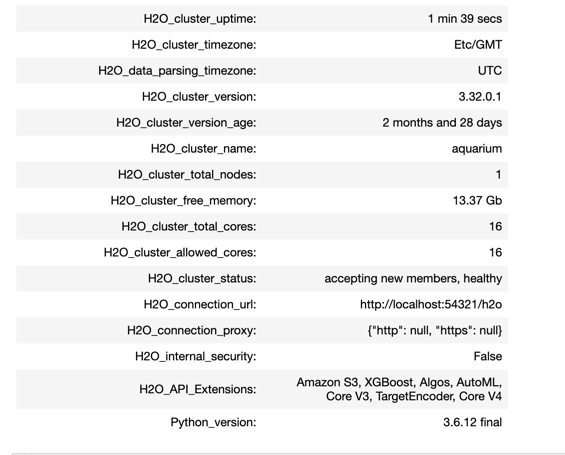If you are working on your machine, if you click on the link above the cluster information, it will take you to your Flow instance, where you can see your models, data frames, plots, and much more. If you are working on Aquarium, go to your lab and click on the Flow URL, and it will take you to your Flow instance. Keep it open on a separate tab, as we will come back to it for Tasks 6 and 7.

Let's import the dataset.

``````loan_level = h2o.import_file("https://s3.amazonaws.com/data.h2o.ai/H2O-3-Tutorials/loan_level_50k.csv")
``````

You will notice that instead of using a subset of the dataset with 500k rows, we are using a subset with 50k rows. We decided to use a smaller dataset to run AutoML for a shorter amount of time. If you would like, you can repeat this self-paced course after you have completed it, and use the same subset that we used in the previous two self-paced courses; just keep in mind that you will need to run AutoML for a much longer time. Before we continue, let's explore some concepts about AutoML that will be useful in this self-paced course.

In this self-paced course, we are using a smaller subset of the Freddie Mac Single-Family dataset compared to the past two self-paced courses. If you have not done so, complete Introduction to Machine Learning with H2O-3 - Classification and Introduction to Machine Learning with H2O-3 - Regression as this self-paced course is a continuation of both of them.

We will use H2O AutoML to make the same predictions as in the previous two self-paced courses:

• Predict whether a mortgage loan will be delinquent or not
• Predict the interest rate for each loan

Complete this self-paced course to see how we achieved those results.

We will start by importing the H2O library that we will use:

``````# Import the required libraries
library(h2o)
``````

``````# Initialize the H2O-3 cluster
h2o.init(bind_to_localhost = FALSE, context_path = "h2o")
``````

If your instance was successfully initialized, you will see a table with a description of it as shown below.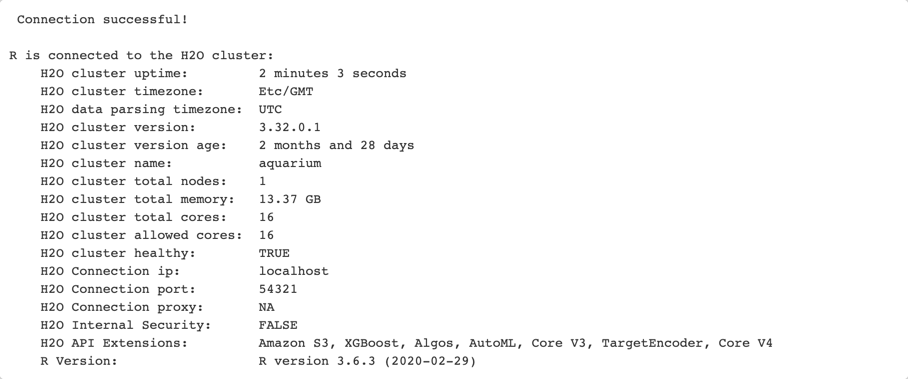If you are working on your machine, if you click on the link above the cluster information, it will take you to your Flow instance, where you can see your models, data frames, plots, and much more. If you are working on Aquarium, go to your lab and click on the Flow URL, and it will take you to your Flow instance. Keep it open on a separate tab, as we will come back to it for Tasks 6 and 7.

Before we import the dataset, let's turn off the progress bar for readability purposes:

``````# Turn off progress bars for notebook readability
h2o.no_progress()
``````

Now, import the dataset

``````# Import dataset
loan_level <- h2o.importFile(path = "https://s3.amazonaws.com/data.h2o.ai/H2O-3-Tutorials/loan_level_50k.csv")
``````

You will notice that instead of using a subset of the dataset with 500k rows, we are using a subset with 50k rows. We decided to use a smaller dataset to run AutoML for a shorter amount of time. If you would like, you can repeat this self-paced course after you have completed it, and use the same subset that we used in the previous two self-paced courses; just keep in mind that you will need to run AutoML for a much longer time

Before we continue, let's explore some concepts about AutoML that will be useful in this self-paced course.

## AutoML

Choosing the best machine learning models and tuning them can be time consuming and exhaustive. Often, it requires levels of expertise to know what parameters to tune. The field of AutoML focuses on solving this issue. AutoML is useful both for experts, by automating the process of choosing and tuning a model; and for non-experts as well, by helping them to create high performing models in a short time frame. Some of the aspects of machine learning that can be automated include data preparation, which can include imputation, one-hot encoding, feature selection/extraction, and also feature engineering. Another aspect that can be automated is the model generation, which includes training a model and tuning it with cartesian or random grid search. Lastly, a third aspect that could be using ensembles, as they usually outperform individual models.

H2O AutoML is an automated algorithm for automating the machine learning workflow, which includes some light data preparation such as imputing missing data, standardization of numeric features, and one-hot encoding categorical features. It also provides automatic training, hyper-parameter optimization, model search, and selection under time, space, and resource constraints. H2O's AutoML further optimizes model performance by stacking an ensemble of models. H2O AutoML trains one stacked ensemble based on all previously trained models and another one on the best model of each family.

The current version of AutoML trains and cross-validates the following model: GLMs, a Random Forest, an Extremely-Randomized Forest, a random grid of Gradient Boosting Machines (GBMs), XGBoosts, a random grid of Deep Neural Nets, and a Stacked Ensemble of all the models. If you would like to know more details about the models trained by AutoML, please visit Which models are trained in the AutoML process? under the FAQ of the AutoML Documentation section. To see how H2O AutoML performs compared to other AutoML algorithms, please look at An Open Source AutoML Benchmark

## Stacked Ensembles

Ensemble machine learning methods use multiple learning algorithms to obtain better predictive performance than the ones that could be obtained from any of the constituent learning algorithms. Many of the popular modern machine learning algorithms are actually ensembles. For example, Random Forest and Gradient Boosting Machine (GBM) are both ensemble learners. Both bagging (e.g., Random Forest) and boosting (e.g., GBM) are methods for ensembling that take a collection of weak learners (e.g., decision tree) and form a single, strong learner.

H2O's Stacked Ensemble method is a supervised ensemble machine learning algorithm that finds the optimal combination of a collection of prediction algorithms using a process called stacking. Like all supervised models in H2O, Stacked Ensemble supports regression, binary classification, and multiclass classification. If you would like to know more, make sure to check the Stacked Ensemble Section in the H2O-3 Documentation.

You can make sure that your dataset was properly imported by printing the first ten rows of your dataset (output not shown here)

``````loan_level.head()
``````

And also, you can look at the statistical summary of it by printing a description of it (output not shown here)

``````loan_level.describe()
``````

We will not focus on the visualization of the dataset as a whole, as we have already worked with this dataset. But, we are going to take a look at the distribution of our response variables.

Let's take a look at the `DELINQUENT` column, which is the response for our classification problem.

``````loan_level["DELINQUENT"].table()
``````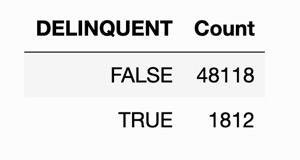As we saw in the classification self-paced course, the dataset is highly imbalanced, which is the same scenario in this case.

Now, let's take a quick look at the response for our regression use-case.

``````loan_level["ORIGINAL_INTEREST_RATE"].hist()
``````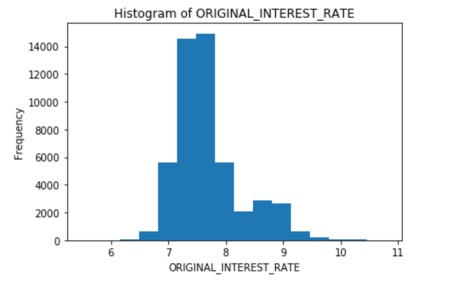Again, we see that the average interest rate ranges from 7% to 8%, similar to what we saw in the previous self-paced course.

Now that we have an idea of the distribution of the responses let's split the dataset. For this self-paced course, we will take a slightly different approach. Instead of splitting the dataset into three sets, we are just going to do 2, a train and test set. We will be using cross-validation to validate our models, as we need to use the k-fold cross-validation in order to get the stacked ensembles from the AutoML.

``````train, test = loan_level.split_frame([0.8], seed=42)
``````

Now, check that the split is what we expected.

``````print("train:%d test:%d" % (train.nrows, test.nrows))
``````

Output:

``````train:39984 test:9946
``````

Since we will need two different responses and predictors, we will do that in each of the corresponding tasks.

You can make sure that your dataset was properly imported by printing the first ten rows of your dataset (output not shown here)

``````# Get first 10 rows and a statistical summary of the data
``````

And also, you can look at the statistical summary of it by printing a description of it (output not shown here)

``````h2o.describe(loan_level)
``````

We will not focus on the visualization of the dataset as a whole, as we have already worked with this dataset. But, we are going to take a look at the distribution of our response variables.

Let's take a look at the `DELINQUENT,` which is the response of our classification problem.

``````# Print a table with the distribution for column "DELINQUENT"
h2o.table(loan_level[, c("DELINQUENT")])
``````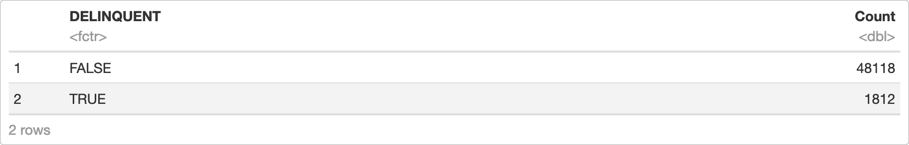As we saw in the classification self-paced course, the dataset is highly imbalanced, which is the same scenario in this case.

Now, let's take a quick look at the response for our regression use-case.

``````# Plot a histogram for the Regression problem target column
h2o.hist(loan_level[, c("ORIGINAL_INTEREST_RATE")])
``````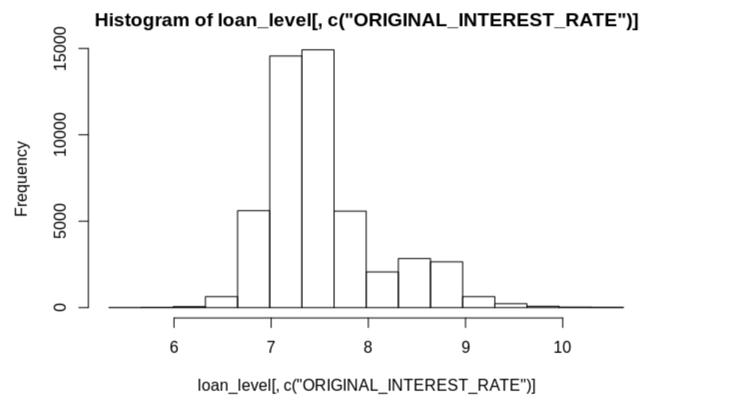Again, we see that the average interest rate ranges from 7% to 8%, similar to what we saw in the previous self-paced course.

Now that we have an idea of the distribution of the responses let's split the dataset. For this self-paced course, we will take a slightly different approach. Instead of splitting the dataset into three sets, we are just going to do 2, a train and test set. We will be using cross-validation to validate our models, as we need to use the k-fold cross-validation in order to get the stacked ensembles from the AutoML.

``````# Split your data into 3 and save into variable "splits"
splits <- h2o.splitFrame(loan_level, c(0.8), seed = 42)

# Extract all 3 splits into train, valid and test
train <- splits[]
test  <- splits[]
``````

Now, check that the split is what we expected.

``````# Check the dimensions of both sets
dim(train)
dim(test)
``````

Output:

``````39984    27

9946   27
``````

Since we will need two different responses and predictors, we will choose them in their corresponding tasks.

We already have our train and test sets, so we just need to choose our response variable, as well as the predictors. We will do the same thing that we did for the first self-paced course.

``````y = "DELINQUENT"
ignore = ["DELINQUENT",
"PREPAID",
"PREPAYMENT_PENALTY_MORTGAGE_FLAG",
"PRODUCT_TYPE"]
x = list(set(train.names) - set(ignore))
``````

Now we are ready to run AutoML. Below you can see some of the default parameters that we could change for AutoML:

``````H2OAutoML(nfolds=5, max_runtime_secs=3600, max_models=None, stopping_metric='AUTO', stopping_tolerance=None, stopping_rounds=3, seed=None, project_name=None)
``````

As you can see, H2O AutoML is designed to have as few parameters as possible, which makes it very easy to use. For this experiment, we will set values for the maximum runtime, and we will choose fifteen minutes, the seed, and the project name; Also, we will set `max_models = 25` so that AutoML runs for fifteen minutes or only trains twenty-five models. Lastly, we will set `sort_metric` to `AUC` so that the leaderboard gets sorted by AUC.

``````aml = H2OAutoML(max_runtime_secs = 900,
max_models = 25,
seed = 42,
project_name='classification',
sort_metric = "AUC")

%time aml.train(x = x, y = y, training_frame = train)
``````

The only required parameters for H2O's AutoML are, `y` `training_frame,` and `max_runtime_secs,` which let us train AutoML for ‘x' amount of seconds and/or `max_models,` which would train a maximum number of models. Please note that `max_runtime_secs` has a default value, while `max_models` does not. The seed is the usual parameter that we set for reproducibility purposes. We also need a project name because we will do both classification and regression with AutoML. Lastly, we are using the default number of folds for cross-validation.

The second line of code has the parameters that we need in order to train our model. For now, we will just pass x, y, and the training frame. Please note that the parameter `x` is optional because if you use all the columns in your dataset, you do not need to declare this parameter. The `leaderboard frame` can be used to score and rank models on the leaderboard, but we will use the validation scores to do so because we will check the performance of our models with the test set.

Below is a list of optional parameters that the user could set for H2O's AutoML:

• validation_frame
• blending_frame
• fold_column
• weights_column
• ignored_columns
• class_sampling_factors
• max_after_balance_size
• max_runtime_secs_per_model
• sort_metric
• exclude_algos
• include_algos
• keep_cross_validation_predictions
• keep_cross_validation_models
• keep_cross_validation_fold_assignment
• verbosity
• export_checkpoints_dir

To learn more about each of them, make sure to check the AutoML Section in the Documentation. We will be using some of them in the regression part of this self-paced course.

Once AutoML is finished, print the leaderboard, and check out the results.

``````lb = aml.leaderboard
``````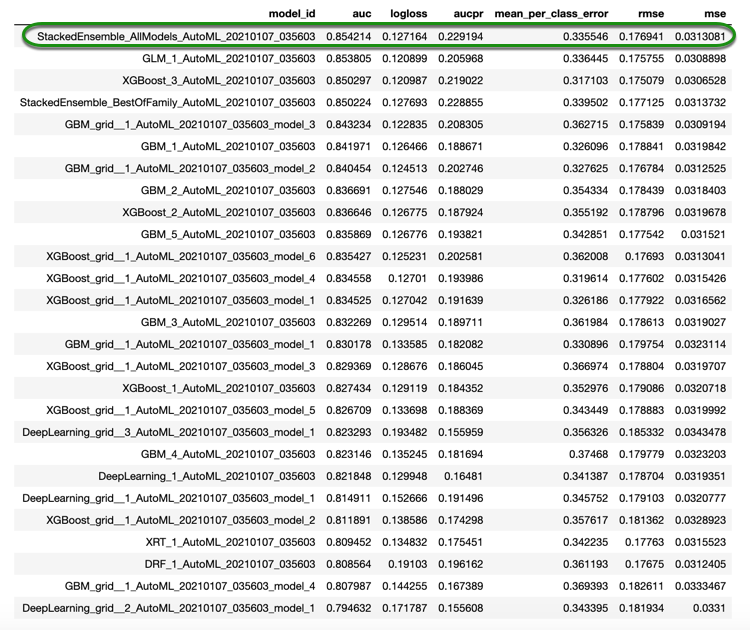Note: We can just print the leaderboard by running `aml.leaderboard`, but we have added an extra line of code just so that get all the models that were scored.

We can also print a leaderboard with two extra columns, one column with the training time, and the second one with the prediction time per row of each model (both columns are printed in milliseconds):

``````lb2 = get_leaderboard(aml, extra_columns = 'ALL')
``````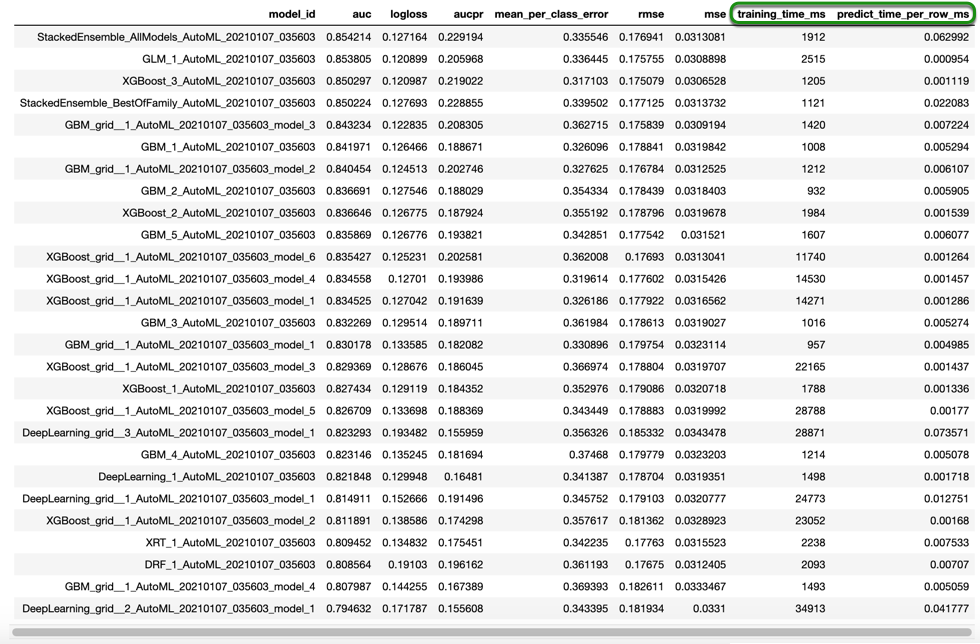By looking at the leaderboard, we can see that the best model is the Stacked Ensemble with all the models, meaning that this Stacked Ensemble was built using all the models that were trained. Secondly, we have the Stacked Ensemble with the best models from each family. The Best of Family Ensemble will usually have a GLM, a Distributed Random Forest, Extremely-Randomized Forest, a GBM, and XGBoost, and Deep Learning model if you give it enough time to train all those models.

Now, let's see how the best model performs on our test set.

``````aml.leader.model_performance(test_data=test)
``````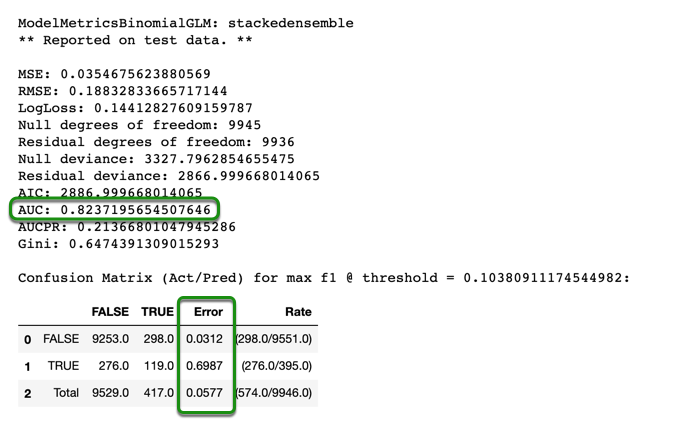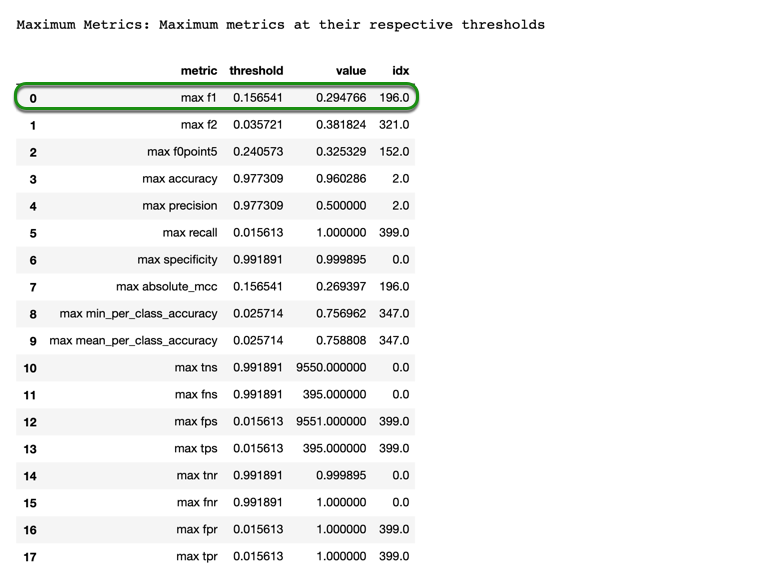By looking at the results, we can see that in fifteen minutes, and with less data, AutoML obtained scores close to what we obtained in the first self-paced course. The AUC that we obtained was 0.824. Although this is could be a good AUC, because we have a very imbalanced dataset, we must also look at the misclassification errors for both classes. As you can see, our model is having a hard time classifying bad loans; this is mainly due because only about 3.6% of loans are labeled as bad loans. However, the model is doing very well when classifying good loans; although it is still far from being the best model, this gives us a solid starting point.

We can also take a quick look at the ROC curve:

``````%matplotlib inline
``````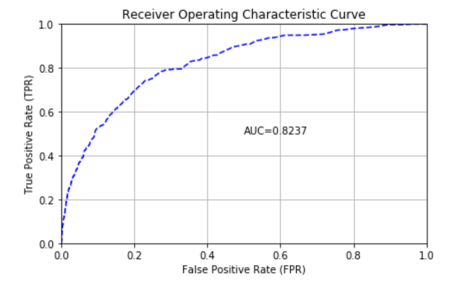As you can see, it's the same AUC value that we obtained in the model summary, but the plot helps us visualize it better.

Lastly, let's make some predictions on our test set.

``````aml.predict(test)
``````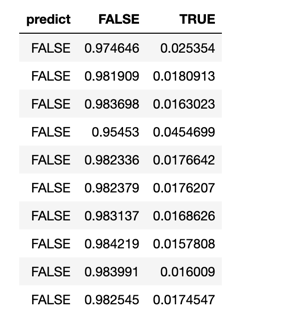As we mentioned in the first self-paced course, the predictions we get are based on a probability. In the frame above, we have a probability for FALSE, and another one for TRUE. The prediction, predict, is based on the threshold that maximizes the F1 score. For example, the threshold that maximizes the F1 is about `0.1061`, meaning that if the probability of TRUE is greater than the threshold, the predicted label would be TRUE.

After exploring the results for our classification problem, let's use AutoML to explore a regression use-case.

We already have our train and test sets, so we just need to choose our response variable, as well as the predictors. We will do the same thing that we did for the first self-paced course.

``````# Identify predictors and response for the classification use case
ignore <- c("DELINQUENT", "PREPAID", "PREPAYMENT_PENALTY_MORTGAGE_FLAG", "PRODUCT_TYPE")
y <- "DELINQUENT"

x <- setdiff(colnames(train), ignore)
``````

Now we are ready to run AutoML. Below you can see some of the default parameters that we could change for AutoML:

``````H2OAutoML(nfolds=5, max_runtime_secs=3600, max_models=None, stopping_metric='AUTO', stopping_tolerance=None, stopping_rounds=3, seed=None, project_name=None)
``````

As you can see, H2O AutoML is designed to have as few parameters as possible, which makes it very easy to use. For this experiment, we will set values for the maximum runtime, and we will choose fifteen minutes, the seed, and the project name; Also, we will set `max_models = 25` so that AutoML runs for fifteen minutes or only trains twenty-five models. Lastly, we will set `sort_metric` to `AUC` so that the leaderboard gets sorted by AUC.

``````# Run AutoML for 25 base models or 15 minutes
aml_cl <- h2o.automl(x = x,
y = y,
training_frame = train,
max_runtime_secs = 900,
max_models = 25,
seed = 42,
project_name = "classification2",
sort_metric = "AUC")
``````

The only required parameters for H2O's AutoML are, `y` `training_frame,` and `max_runtime_secs,` which let us train AutoML for ‘x' amount of seconds and/or `max_models,` which would train a maximum number of models. Please note that `max_runtime_secs` has a default value, while `max_models` does not. The seed is the usual parameter that we set for reproducibility purposes. We also need a project name because we will do both classification and regression with AutoML. Lastly, we are using the default number of folds for cross-validation.

The second line of code has the parameters that we need in order to train our model. For now, we will just pass x, y, and the training frame. Please note that the parameter `x` is optional because if you were using all the columns in your dataset, you would not need to declare this parameter. The `leaderboard frame` can be used to score and rank models on the leaderboard, but we will use the validation scores to do so because we will check the performance of our models with the test set.

Below is a list of optional parameters that the user could set for H2O's AutoML:

• validation_frame
• blending_frame
• fold_column
• weights_column
• ignored_columns
• class_sampling_factors
• max_after_balance_size
• max_runtime_secs_per_model
• sort_metric
• exclude_algos
• include_algos
• keep_cross_validation_predictions
• keep_cross_validation_models
• keep_cross_validation_fold_assignment
• verbosity
• export_checkpoints_dir

To learn more about each of them, make sure to check the AutoML Section in the Documentation. We will be using some of them in the regression part of this self-paced course.

Once AutoML is finished, print the leaderboard, and check out the results.

``````# View the AutoML Leaderboard
``````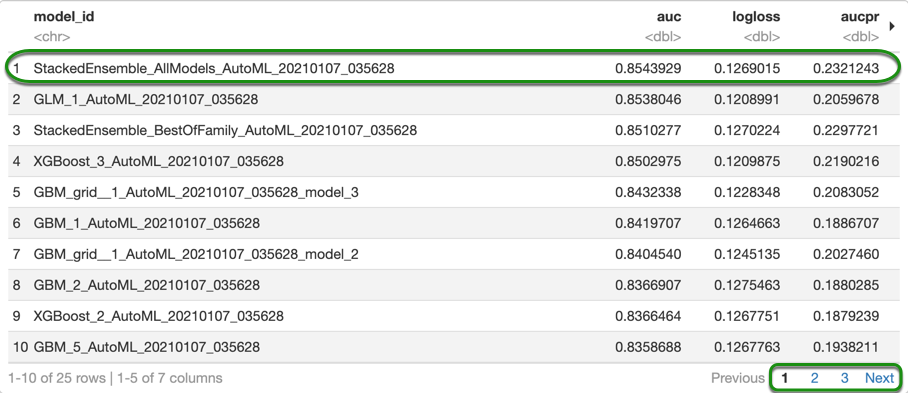Note: We can just print the leaderboard by running `aml.leaderboard`, but we have added an extra line of code just so that we get all the models that were scored. Also, note that you can view the other models by clicking Next or any of the numbers at the bottom left of the table. And you can view the other metrics for the models by clicking the arrow at the top right corner of the table.

We can also print a leaderboard with two extra columns, one column with the training time, and the second one with the prediction time per row of each model (both columns are printed in milliseconds):

``````# Get leaderboard with 'extra_columns = 'ALL'
lb2 <- h2o.get_leaderboard(aml_cl, extra_columns = "ALL")
``````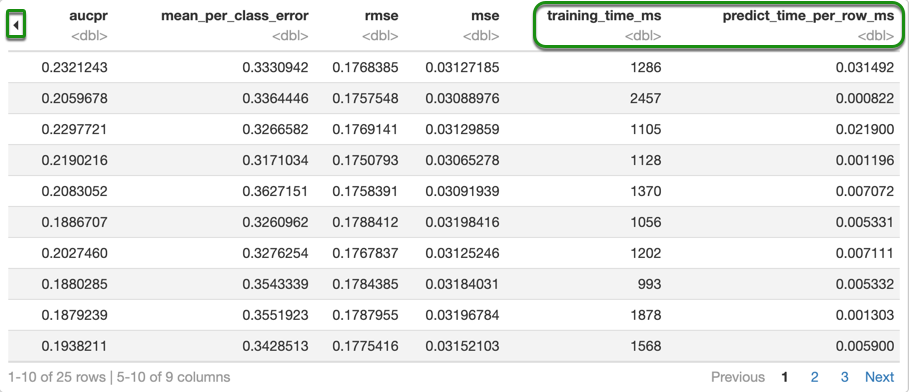Note: In order to show the training time, and predict time per row, only the second half of the table is being shown.

By looking at the leaderboard, we can see that the best model is the Stacked Ensemble with all the models, meaning that this Stacked Ensemble was built using all the models that were trained. Secondly, we have the Stacked Ensemble with the best models from each family. The Best of Family Ensemble will usually have a GLM, a Distributed Random Forest, Extremely-Randomized Forest, a GBM, and XGBoost, and Deep Learning model if you give it enough time to train all those models.

Now, let's see how the best model performs on our test set.

``````# Save the test performance of the leader model
aml_cl_test_perf
``````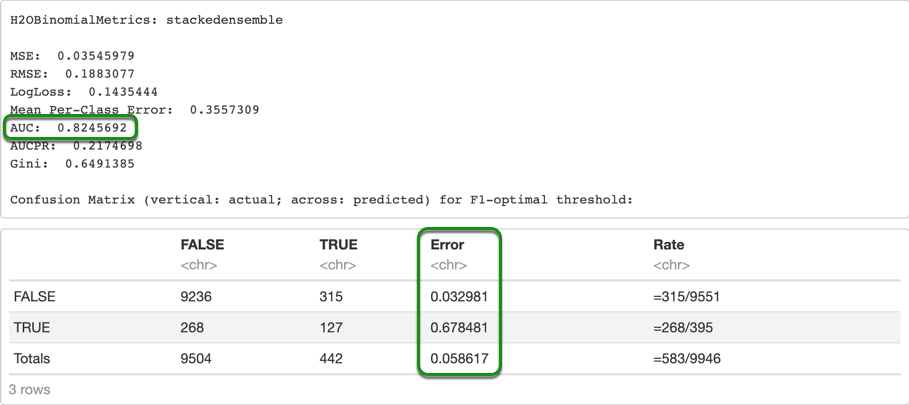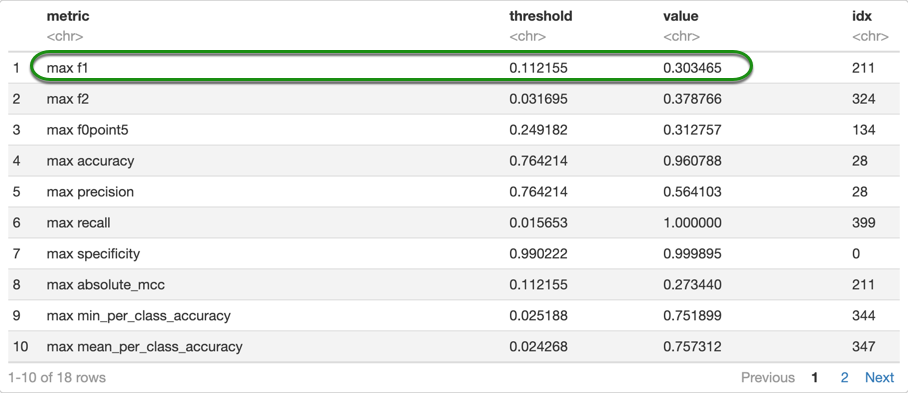By looking at the results, we can see that in fifteen minutes, and with less data, AutoML obtained scores somewhat close to what we obtained in the first self-paced course. The AUC that we obtained was 0.823. Although this is a good AUC, because we have a very imbalanced dataset, we must also look at the misclassification errors for both classes. As you can see, our model is having a hard time classifying bad loans; this is mainly due because only about 3.6% of loans are labeled as bad loans. However, the model is doing very well when classifying good loans; although it is still far from being the best model, this gives us a solid starting point.

We can also take a quick look at the ROC curve:

``````%matplotlib inline
``````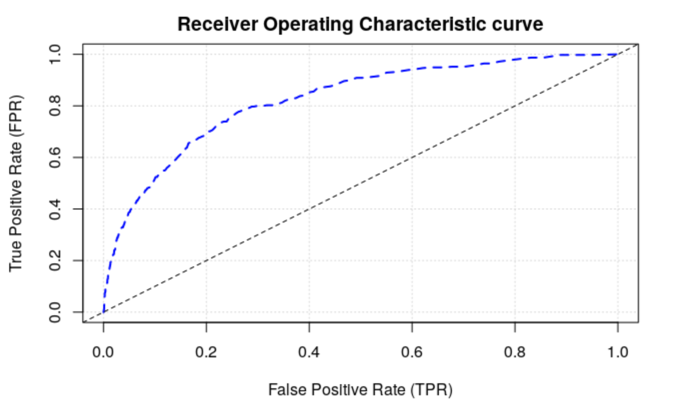As you can see, it's the same AUC value that we obtained in the model summary, but the plot helps us visualize it better.

Lastly, let's make some predictions on our test set.

``````# Make predictions
``````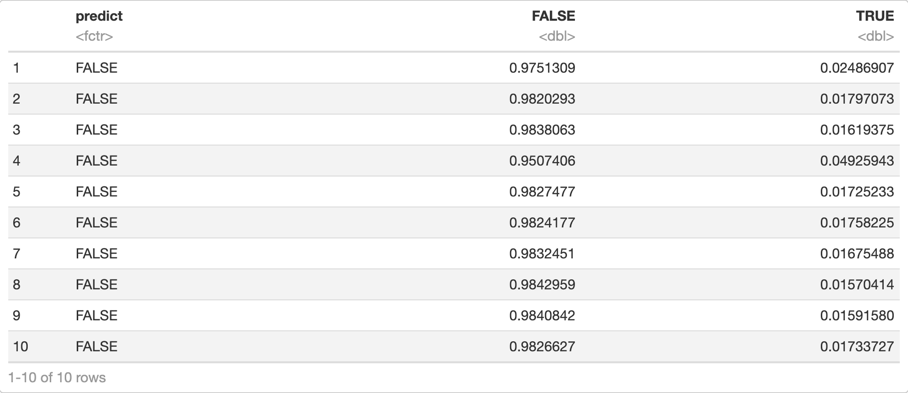As we mentioned in the first self-paced course, the predictions we get are based on a probability. In the frame above, we have a probability for FALSE, and another one for TRUE. The prediction, predict, is based on the threshold that maximizes the F1 score. For example, the threshold that maximizes the F1 is about `0.1116` (this value comes from the maximun metrics table) meaning that if the probability of TRUE is greater than the threshold, the predicted label would be TRUE.

After exploring the results for our classification problem, let's use AutoML to explore a regression use-case.

For our regression use-case, we are using the same dataset and the same training and test sets. But we do need to choose our predictors and response columns, and we will do it as follow:

``````y_reg = "ORIGINAL_INTEREST_RATE"

ignore_reg = ["ORIGINAL_INTEREST_RATE",
"FIRST_PAYMENT_DATE",
"MATURITY_DATE",
"MORTGAGE_INSURANCE_PERCENTAGE",
"PREPAYMENT_PENALTY_MORTGAGE_FLAG",
"LOAN_SEQUENCE_NUMBER",
"PREPAID",
"DELINQUENT",
"PRODUCT_TYPE"]

x_reg = list(set(train.names) - set(ignore_reg))
``````

You can print both your y and x variables

``````print("y:", y_reg, "\nx:", x_reg)
``````

Now we are ready to start our second AutoML model and train it. This time we will use a time constrain, and set `max_runtime_secs` to 900 seconds, or 15 minutes. You will notice that we are specifying the stopping metric and also the sort metric. In the second self-paced course, we focused on RMSE and MAE to check the performance of our model, and we noticed that the two values seemed very correlated. For that reason, we could use any of those metrics. We will use the RMSE for early stopping because it penalizes the error more than the MAE, and we will also use it to sort the leaderboard based on the best value.

``````aml = H2OAutoML(max_runtime_secs = 900,
seed = 42,
project_name = 'regression',
stopping_metric = "RMSE",
sort_metric = "RMSE")

%time aml.train(x = x_reg, y = y_reg, training_frame = train)
``````

Once it's done, print the leaderboard.

``````lb = aml.leaderboard
``````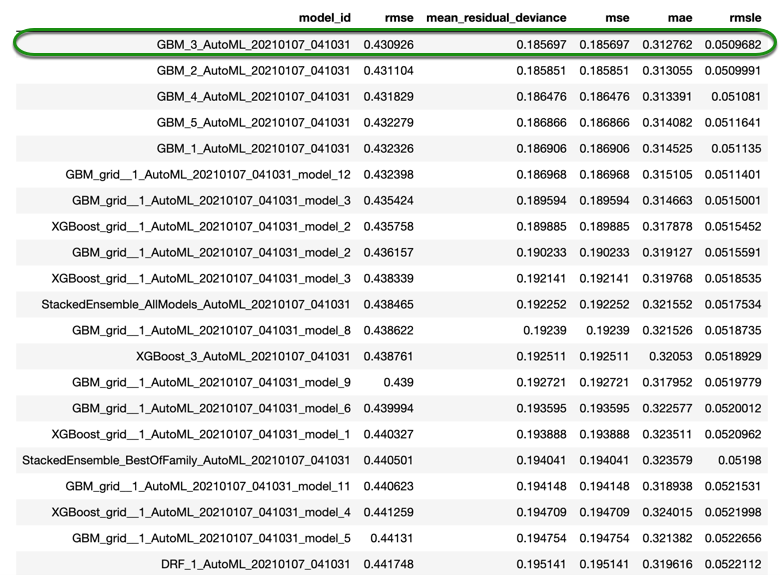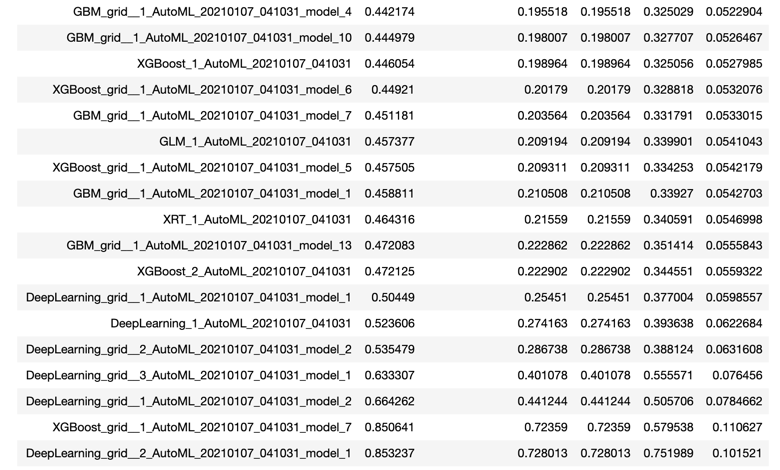The leaderboard shows that the GBM models clearly dominated this task. We can retrieve the best model with the `model.leader` command; but, what if we wanted to get another model from our leaderboard? One way to do so is shown below:

``````# Get model ids for all models in the AutoML Leaderboard

# Get the top GBM model
gbm = h2o.get_model([mid for mid in model_ids if "GBM" in mid])
``````

To retrieve different models, you just need to change the name of the model, `GBM`, to the model that you want. For example, if you wanted to get the best XGBoost in the leaderboard, you would need to make the following change (you do not need to run the following line of code, this is just an example)

``````xgb = h2o.get_model([mid for mid in model_ids if "XGBoost" in mid])
``````

Now that we have retrieved the best model, we can take a look at some of the parameters:

``````print("ntrees = ", gbm.params['ntrees'])
print("max depth = ", gbm.params['max_depth'])
print("learn rate = ", gbm.params['learn_rate'])
print("sample rate = ", gbm.params['sample_rate'])
``````

Output:

``````ntrees =  {'default': 50, 'actual': 63}
max depth =  {'default': 5, 'actual': 8}
learn rate =  {'default': 0.1, 'actual': 0.1}
sample rate =  {'default': 1.0, 'actual': 0.8}
``````

If you want to see a complete list with all the parameters you can use the following command (output not shown here)

``````gbm.params
``````

This is useful because we can see the parameters that were changed and the ones that were kept as default. After exploring those parameters, we could try to tune a GBM with parameters close to the ones from the AutoML model and see if we can get a better score.

Now let's take a quick look at the model summary.

``````gbm
``````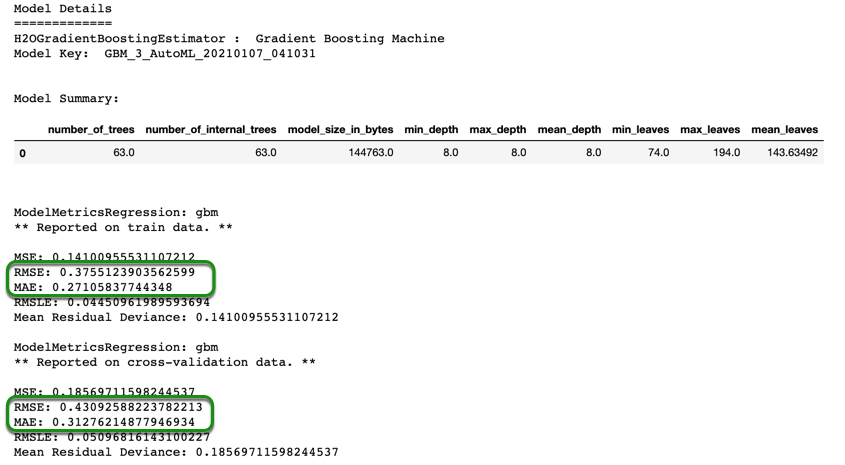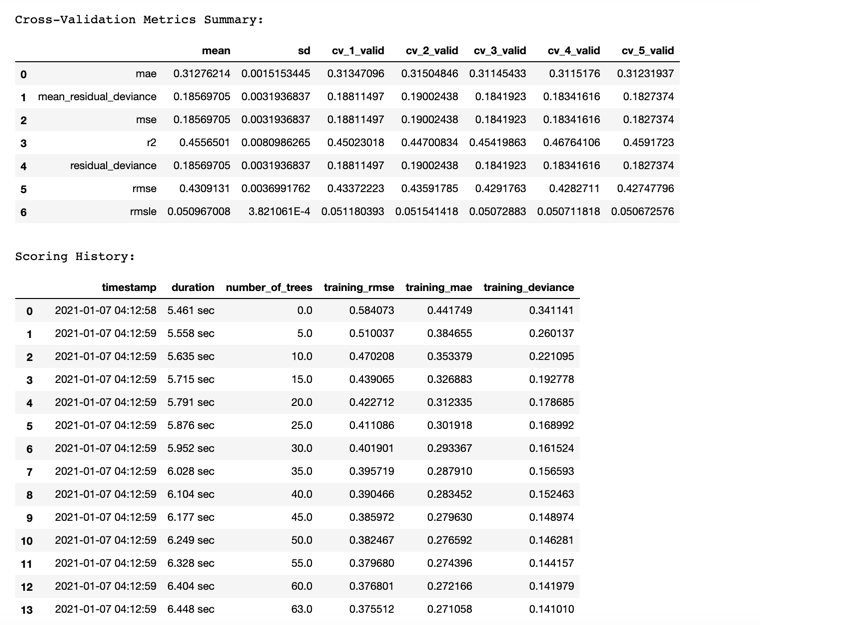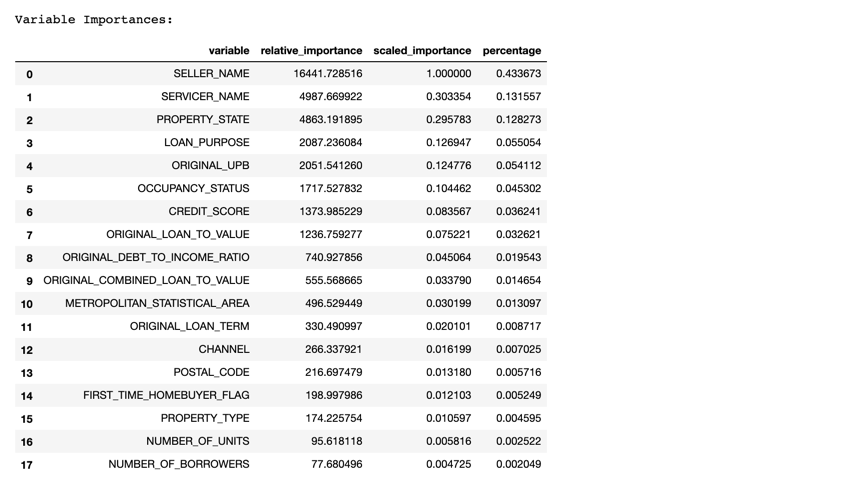In the model summary above, we can see some of the parameters of our model, the metrics on the training data, and also the metrics from the cross-validation, as well as a detailed cross-validation metrics summary. We can also look at scoring history. However, by looking at the RMSE and MAE in the picture above for both training and validation, we can see that the model was starting to overfit because the training error is much lower than the validation error. Lastly, we can see the variable importance table, which shows both relative_importance, as well as scaled_importance, and also the percentage. For this model, the most important variable is `SELLER_NAME`, meaning that for our model, knowing which bank is providing the loan is very important.

Now, let's see how the leader from our AutoML performs on our test set.

``````gbm.model_performance(test_data=test)
``````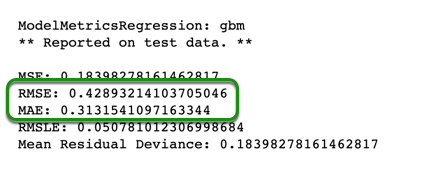In case you had retrieved a different model other than the leader, and you actually wanted to check the performance of the best model in the leaderboard, you can do the following:

``````aml.leader.model_performance(test_data=test)
``````

If you do that right now, you will see the same results because the gbm that we retrieved was the leader.

We can see that the test RMSE and MAE, 0.4289 and 0.3132 respectively, are very close to the validation RMSE and MAE, 0.4309 and 0.3127, which shows us that doing 5-fold cross-validation gives us a good estimation of the error on unseen data.

Now, let's make some predictions on our test set.

``````pred = gbm.predict(test)
pred = pred.cbind(test['ORIGINAL_INTEREST_RATE'])
``````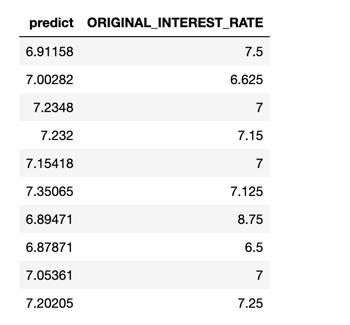Please note that we just combined the response column from our test frame to our predictions to see how the predictions compare to the actual value.

Out of the first ten predictions, most of them are very close to the actual values, with the exception of some predictions, such as the seventh prediction, which is 6.89, compared to the actual value which is 8.75. Because the RMSE is higher than the MAE, we can deduce that we have a couple of instances similar to the one mentioned above, because the larger errors are penalized more.

Now, let's try to do what we just did one more time, but this time in Flow.

For our regression use-case, we are using the same dataset and the same training and test sets. But we do need to choose our predictors and response columns, and we will do it as follow:

``````# Identify predictors and response for the regression use case
ignore_reg <- c("ORIGINAL_INTEREST_RATE", "FIRST_PAYMENT_DATE", "MATURITY_DATE", "MORTGAGE_INSURANCE_PERCENTAGE",
"PREPAYMENT_PENALTY_MORTGAGE_FLAG", "LOAN_SEQUENCE_NUMBER", "PREPAID",
"DELINQUENT", "PRODUCT_TYPE")

y_reg <- "ORIGINAL_INTEREST_RATE"

x_reg <- setdiff(colnames(train), ignore_reg)
x_reg
``````

Now we are ready to start our second AutoML model and train it. This time we will only use a time constrain, and set `max_runtime_secs` to `900 seconds`, or fifteen minutes. You will notice that we are specifying the stopping metric and also the sort metric. In the second self-paced course, we focused on RMSE and MAE to check the performance of our model, and we noticed that the two values seemed very correlated. For that reason, we could use any of those metrics. For this task, we will use the RMSE for early stopping because it penalizes the error more than the MAE, and we will also use it to sort the leaderboard based on the best value.

``````#Run AutoML for 15 minutes
aml_reg <- h2o.automl(x = x_reg,
y = y_reg,
training_frame = train,
max_runtime_secs = 900,
stopping_metric = 'RMSE',
seed = 42,
project_name = "regression",
sort_metric = 'RMSE')
``````

Once it's done, print the leaderboard.

``````# View the AutoML Leaderboard
``````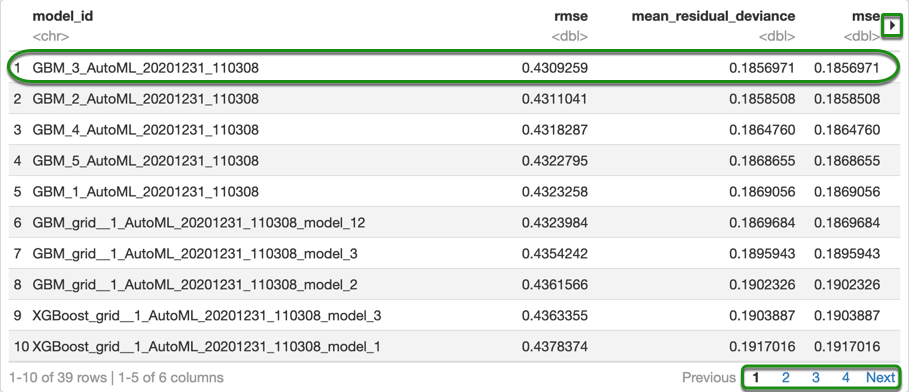Note: In the table above, only the top ten models are shown. You can view the other models by clicking Next in your table.

The leaderboard shows that the GBM models clearly dominated this task. We can retrieve the best model with the `model.leader` command; but, what if we wanted to get another model from our leaderboard? One way to do so is shown below:

``````# Get model ids for all models in the AutoML Leaderboard
# Get top GBM from leaderboard
gbm <- h2o.getModel(grep("GBM", model_ids, value = TRUE))
``````

In case you are trying to retrieve another model, you would need to change the name `GBM`, in the code above, to the name of the model that you want, and that should retrieve the desired model. For example, if you wanted to get the best XGBoost in the leaderboard, you would need to make the following change (you do not need to run the following line of code, this is just an example).

``````xgb <- h2o.getModel(grep("XGBoost", model_ids, value = TRUE))
``````

You could retrieve any model you want by providing a model ID to the `getModel` function as follow:

``````gbm <- h2o.getModel("desired_model_id")
``````

Now that we have retrieved the best model, we can take a look at some of the parameters:

``````# Retrieve specific parameters from the obtained model
gbm@allparameters[["ntrees"]]
gbm@allparameters[["max_depth"]]
gbm@allparameters[["learn_rate"]]
gbm@allparameters[["sample_rate"]]
``````

Output:

``````63
8
0.1
0.8
``````

After exploring those parameters, a next step could be to try to tune the GBM with grid searches around the parameters obtained from AutoML and see if we can get a better score.

Now let's take a quick look at the model summary.

``````# Print the results for the model
gbm
``````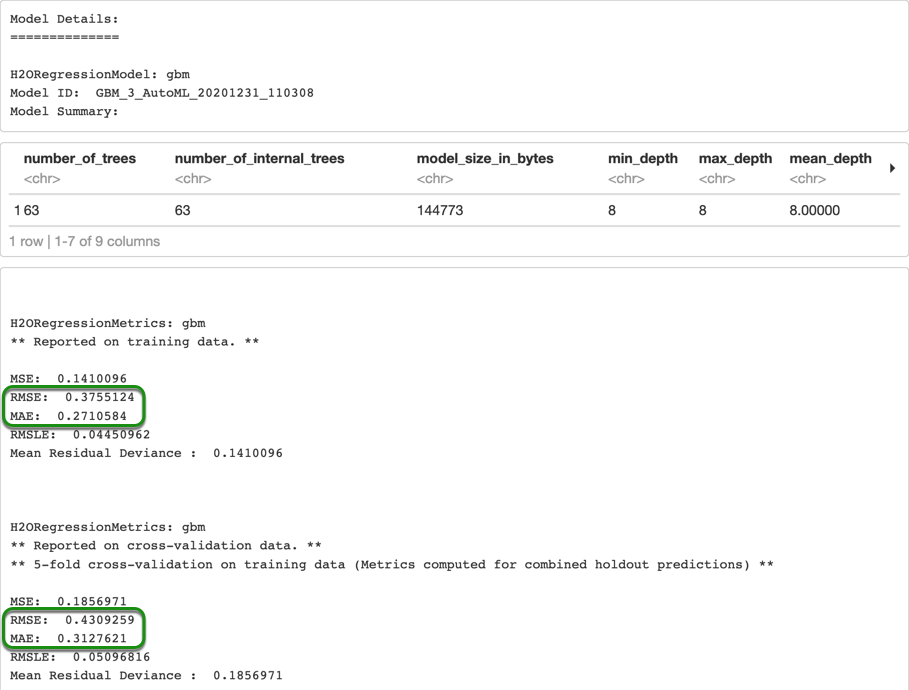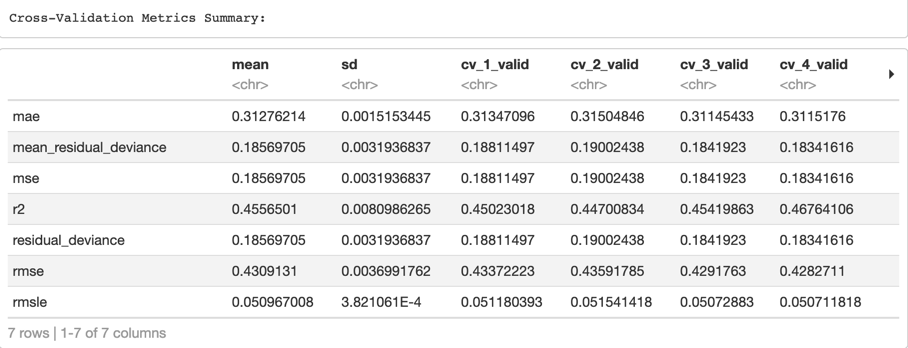In the model summary above, we can see some of the parameters of our model, the metrics on the training data, and also the metrics from the cross-validation, as well as a detailed cross-validation metrics summary. We can also look at scoring history. However, by looking at the RMSE and MAE in the picture above for both training and validation, we can see that the model was starting to overfit because the training error is much lower than the validation error. Lastly, we can see the variable importance table, which shows both relative_importance, as well as scaled_importance, and also the percentage. For this model, the most important variable is `SELLER_NAME`, meaning that for our model, knowing which bank is providing the loan is very important.

Now, let's see how the leader from our AutoML performs on our test set.

``````# Save the test performance of the model
gbm_test_perf <- h2o.performance(gbm, test)
``````

Now you can print the RMSE and the MAE:

``````# Print the test RMSE
h2o.rmse(gbm_test_perf)
``````
``````0.4289321
``````
``````# Print the test MAE
h2o.mae(gbm_test_perf)
``````
``````0.3131541
``````

We can see that the test RMSE and MAE, 0.4289 and 0.3132 respectively, are very close to the validation RMSE and MAE, 0.4309 and 0.3128, which shows us that doing 5-fold cross-validation gives us a good estimation of the error on unseen data.

Now, let's make some predictions on our test set.

``````# Make predictions on test set
gbm_pred <- h2o.predict(gbm, test)

# Combine the predictions with the original response column
preds <- h2o.cbind(test[, c("ORIGINAL_INTEREST_RATE")], gbm_pred)

``````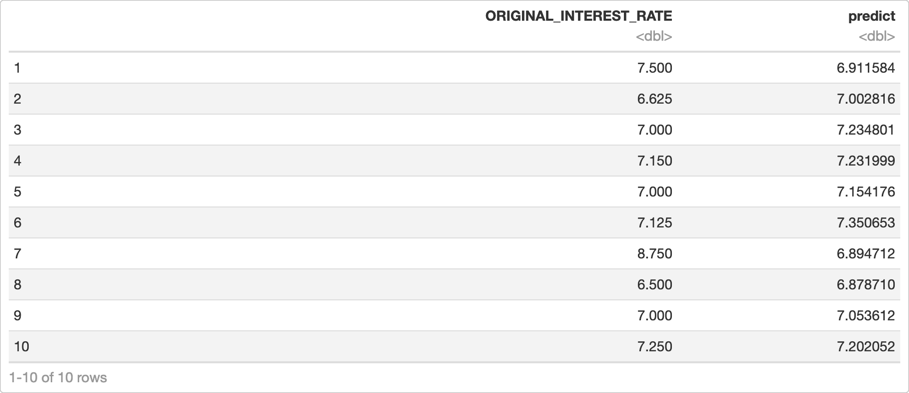Please note that we just combined the response column from our test frame with our predictions to see how the predictions compare to the actual value.

Out of the first ten predictions, most of them are very close to the actual values, with the exception of some predictions, such as the seventh prediction, which is 6.89, compared to the actual value which is 8.75. Because the RMSE is higher than the MAE, we can deduce that we have a couple of instances similar to the one mentioned above, because the larger errors are penalized more.

Now, let's try to do what we just did one more time, but this time in Flow.

Our dataset should already be in our Flow instance; go to your Flow instance, by either clicking the link we mentioned at the beginning of the self-paced course or switching to the tab that you opened earlier.

Your Flow instance should look similar to the one below: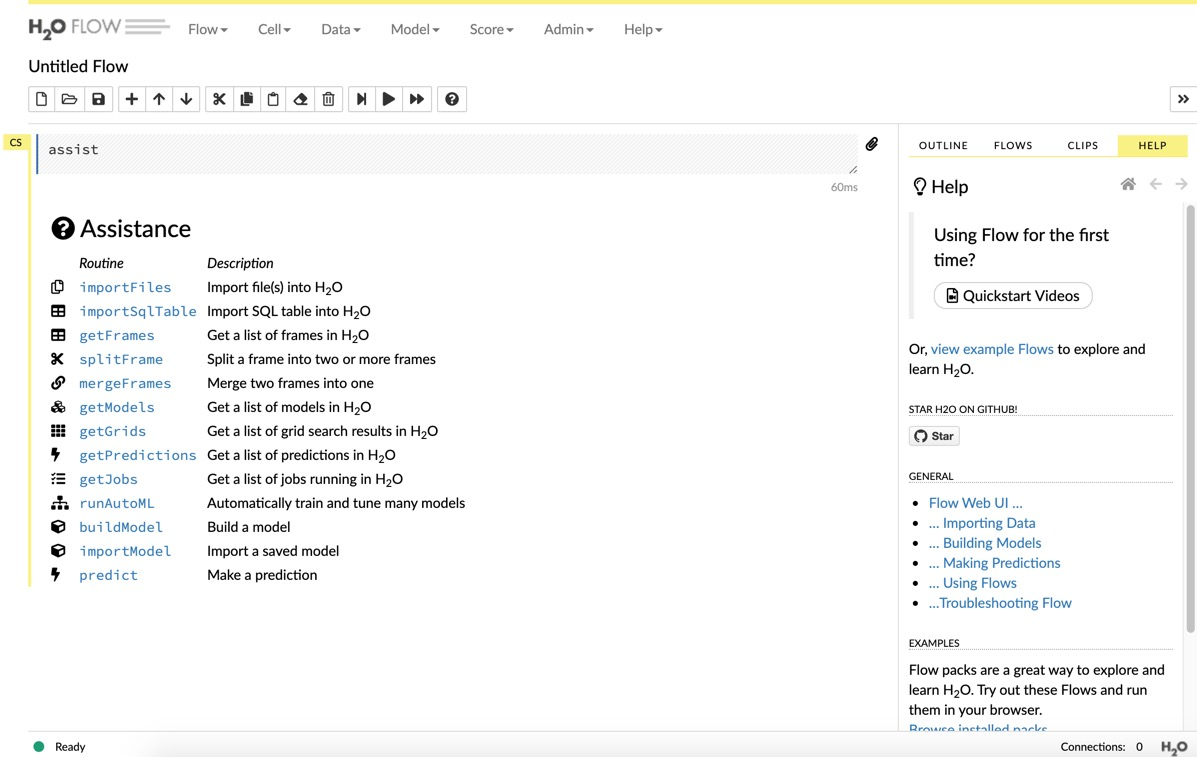Click on `getFrames` in the Assistance panel on the left side. This will show you a list of all the frames currently stored in your instance.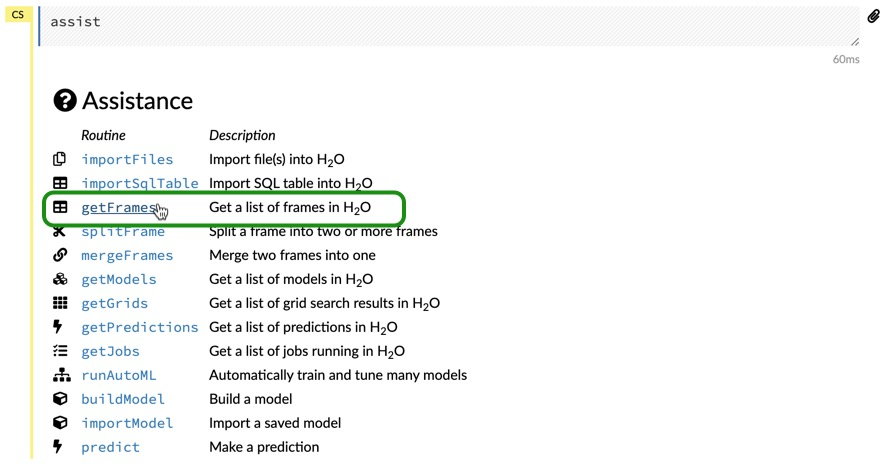Here you will be able to see all the "tables" that we have printed in our Jupyter Notebook, for example, you will see the outputs of the `.head()` function that we used earlier. Also, you will see a frame with the predictions and all the frames which we have worked with. Look for the `loan_level_50k.hex` frame, this is the dataset that we imported at the beginning of the self-paced course. In the picture below, you will see three frames highlighted, the first one is the dataset we imported, and the other two are the train and test sets.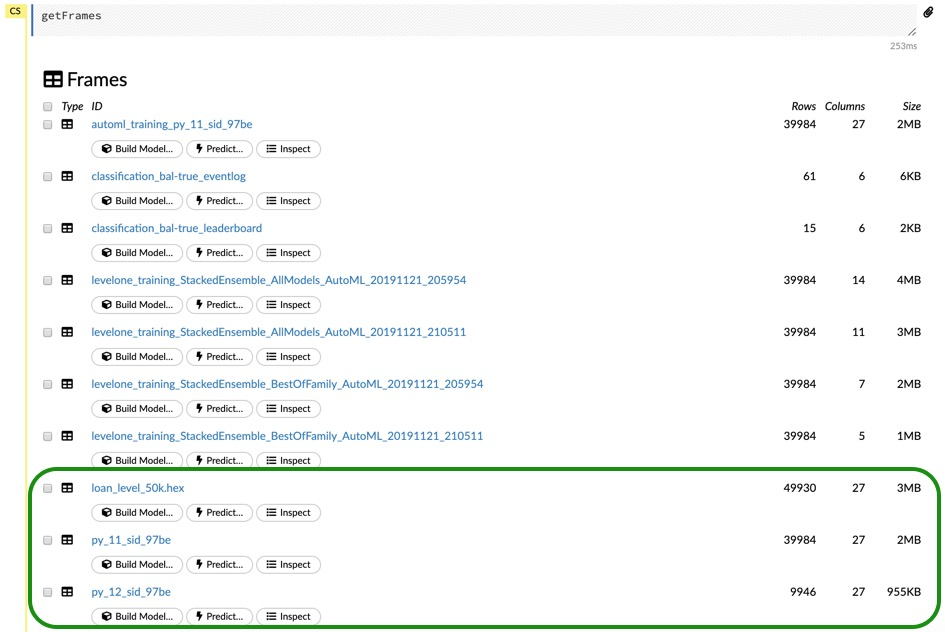To import a file into Flow, you would just need to run the following command in a new cell

``````importFiles ["https://s3.amazonaws.com/data.h2o.ai/DAI-Tutorials/loan_level_50k.csv"]
``````

Note: For a more detailed guide on how to import a file into Flow, check out Task 3 of Introduction to Machine Learning with H2O-3 - Classification.

Even though we already have the train and test set, let's split the loan_level_50k.hex file, that way we can give a specific name to the train and test sets. Use the ratio 0.8 for train and 0.2 for test.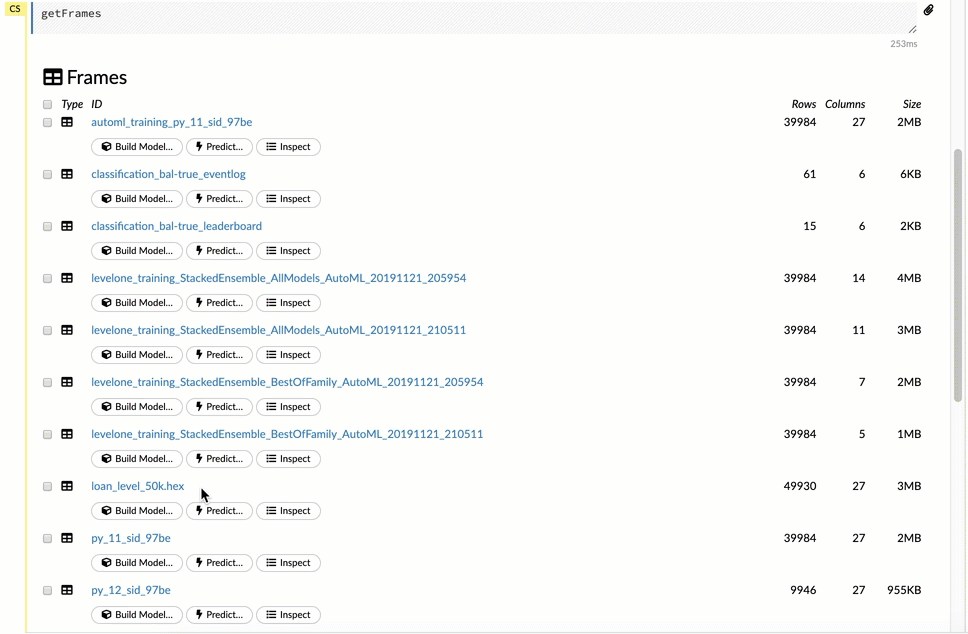Now that we can easily find our train and test set, scroll up again to the Assistance panel and click on `runAutoML`.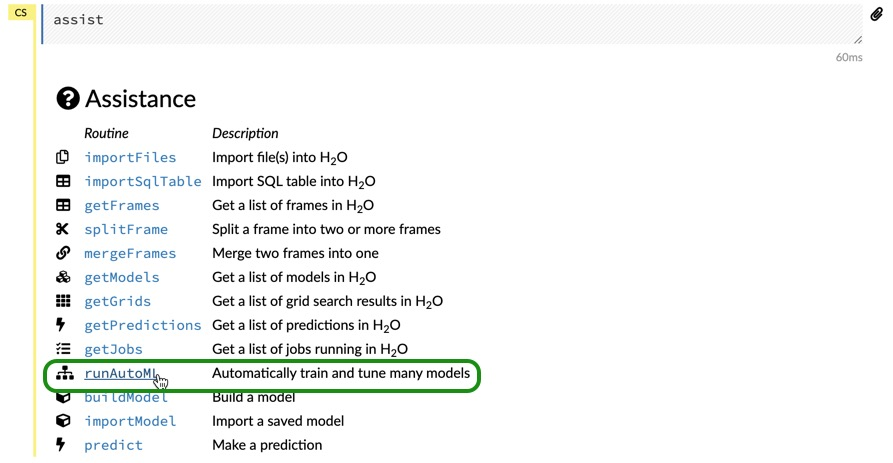You can name your model `flow-automl.` For Training Frame choose `train.` For the Response Column select `DELINQUENT.` For the Leaderboard Frame select `test.`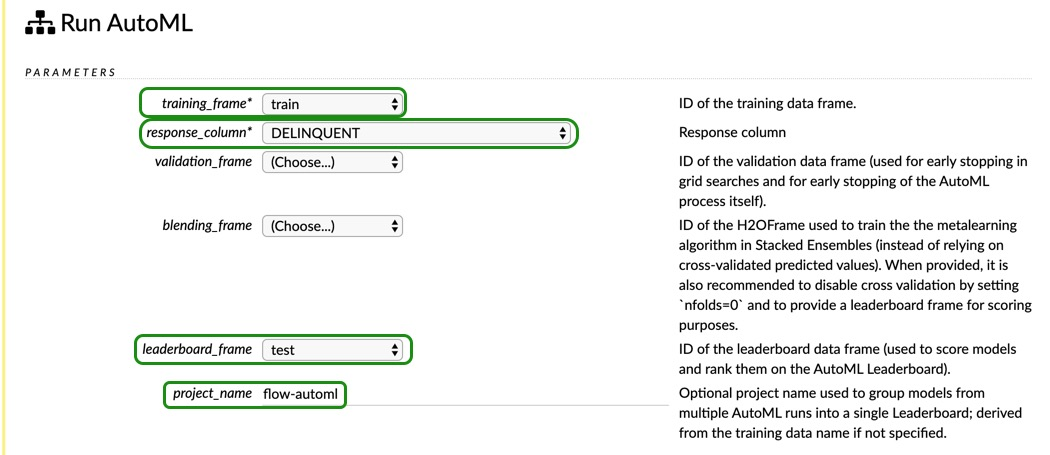Click on the balance_classes box. Now, for Ignored Columns Select

• `PREPAYMENT_PENALTY_MORTGAGE_FLAG`,
• `PRODUCT_TYPE,`
• `PREPAID.`

Also, change the sort metric to `AUC.`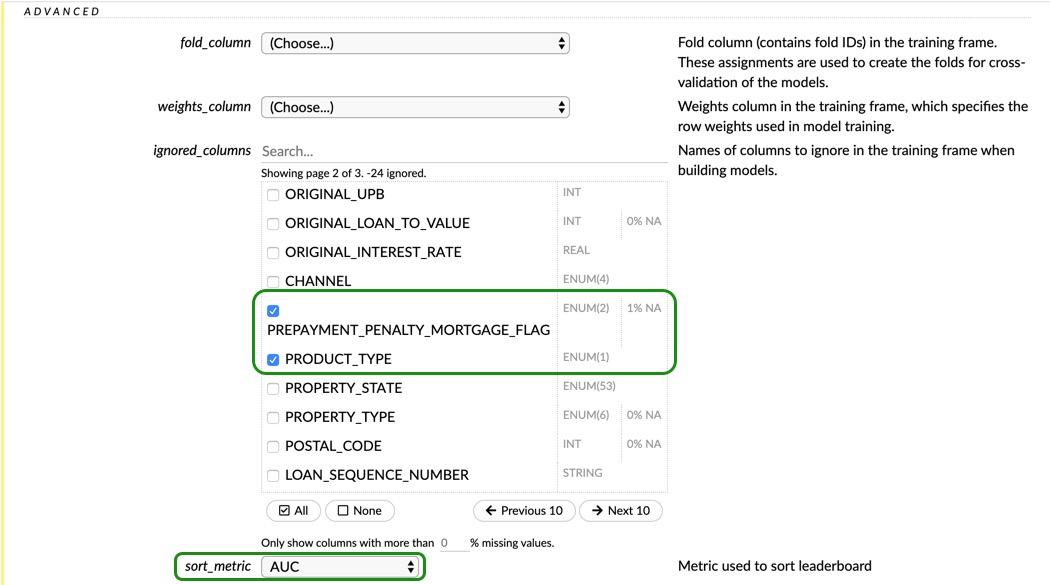Since the Deep Learning models take longer to train, let's exclude it from this AutoML, just check the box next to DeepLearning as shown below. Change the Seed to `42` and Max Run Time (sec) to `900.`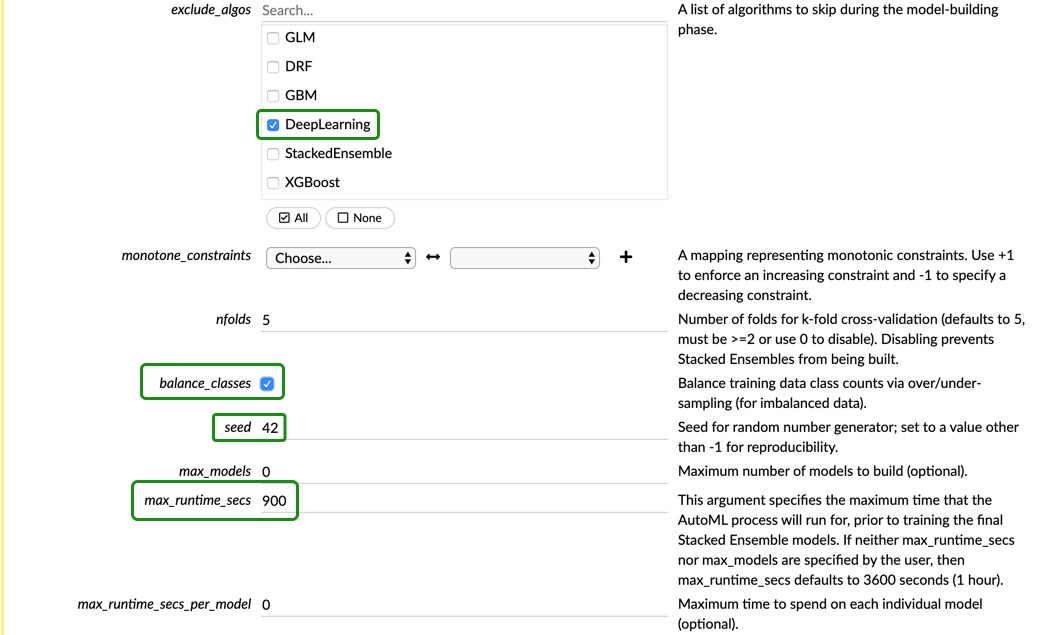Leave the Expert settings as they are, and click on Build Model. Make sure your AutoML settings look similar to the ones shown in the images above.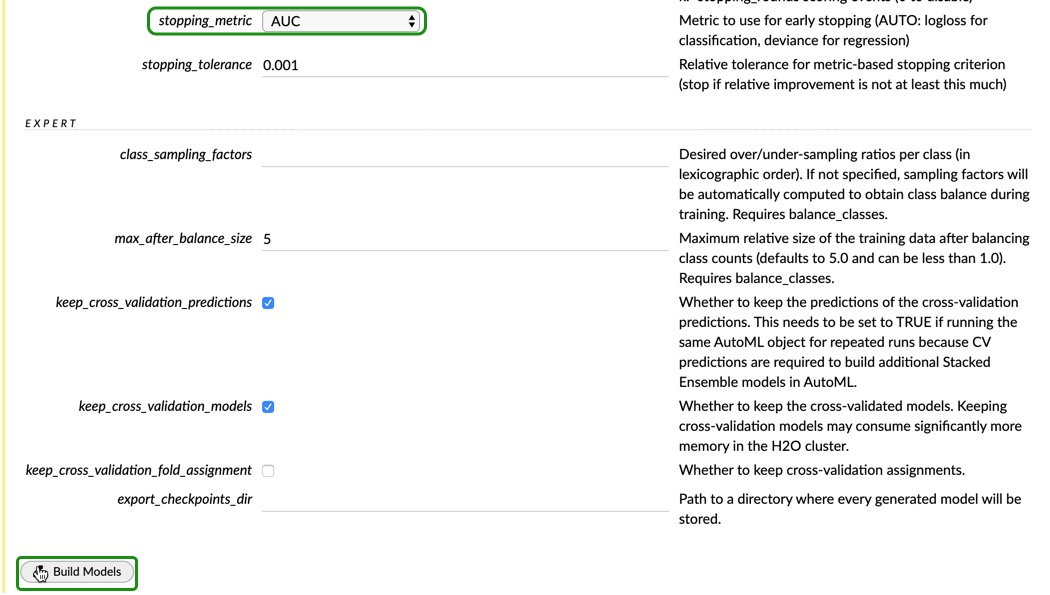Once your model is building, you will see a cell with a dialog similar to the image below: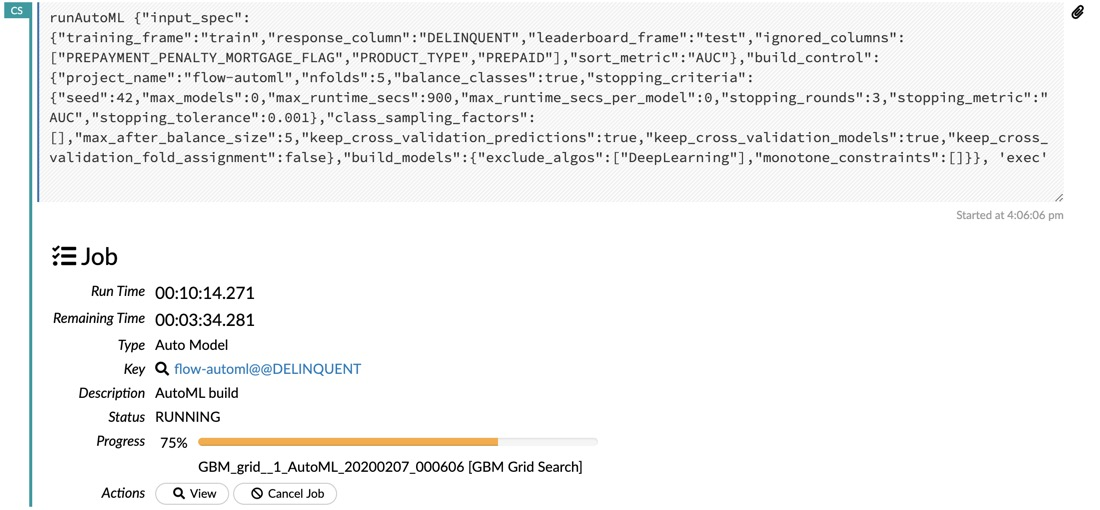Once AutoMl finishes running, click on View, and you will see a new cell that will have the leaderboard and an Event Log tab as shown below: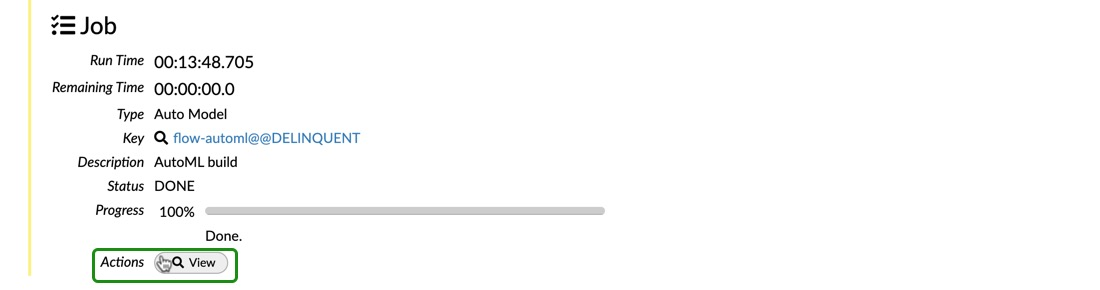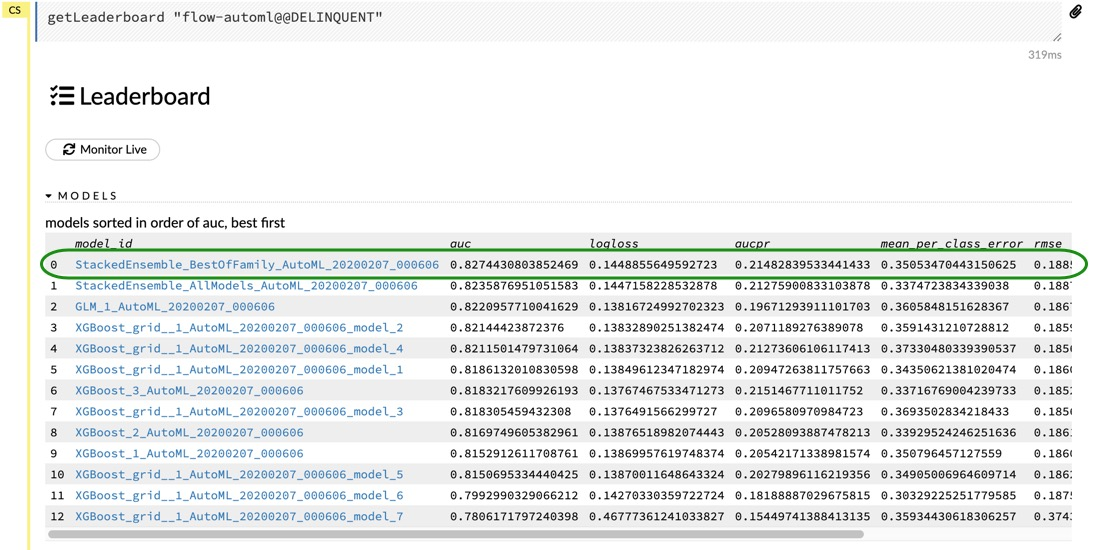In Flow, we can access any of the models in the leaderboard just by clicking on it. Click on the `StackedEnsemble_BestOfFamily` model, and you will get all the details about it. Below you can see all the outputs that Flow shows you from that model. Some of the outputs are the model parameters, the training ROC Curve, the cross-validation ROC curve, and much more.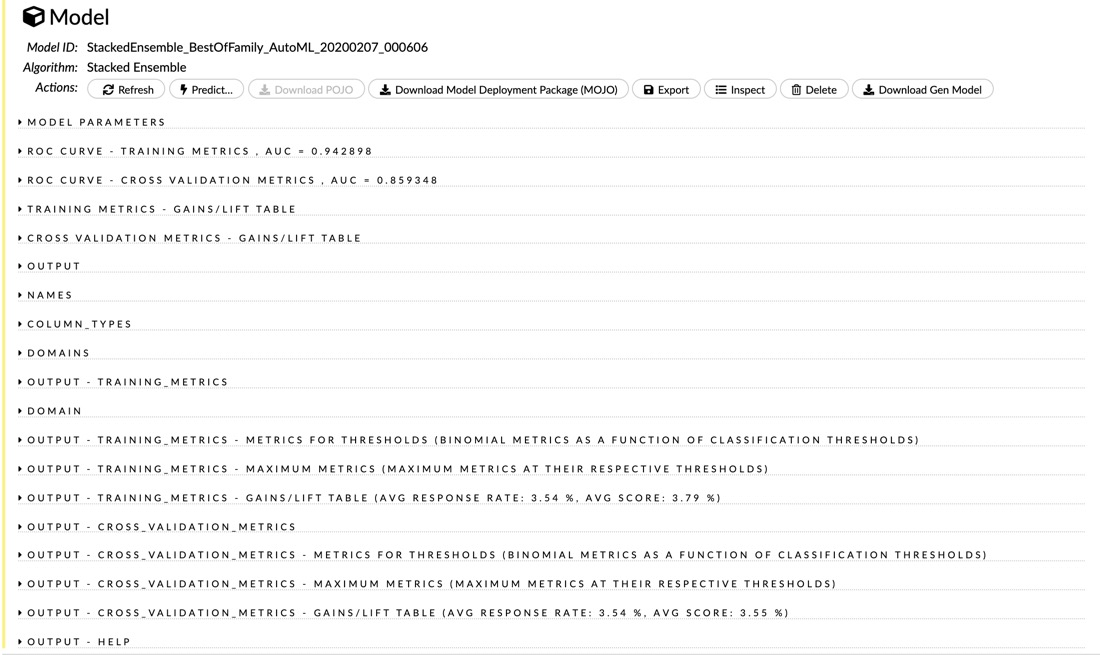Go to the `ROC CURVE - CROSS VALIDATION METRIC` and on the criterion dropdown menu, choose `f1` and you will see something like the image below: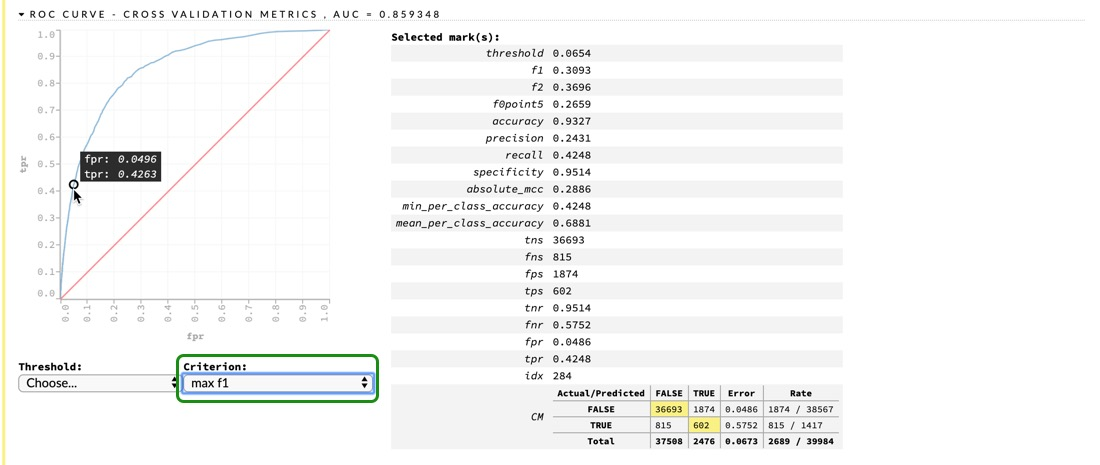You can see all the metrics at the specified threshold, which gives us the max F1 score. You can also see the confusion matrix, and how the misclassification error for the TRUE class seems lower than what we got in the Jupyter Notebook. Now, let's try to make some predictions on our test set. At the top of the cell, click on `Predict`.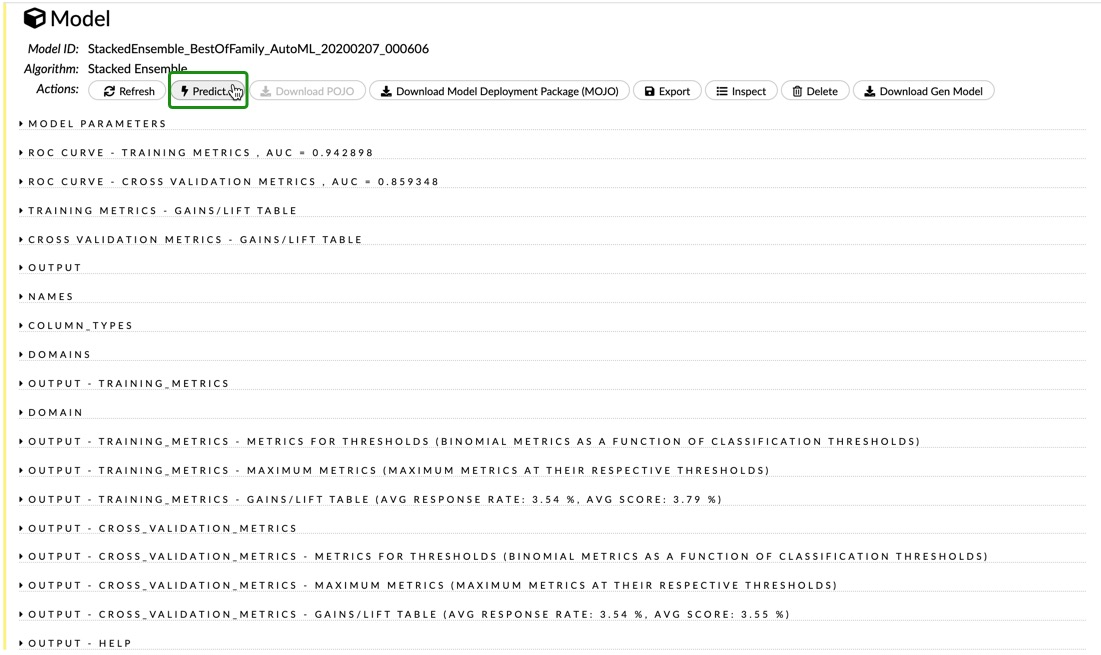You will see another cell that will ask for a name and frame. You can name it `automl-cl-predictions` (cl referring to classifier). And for the Frame click on the dropdown menu, and look for the `test` frame. Once you have updated those two fields, click on Predict.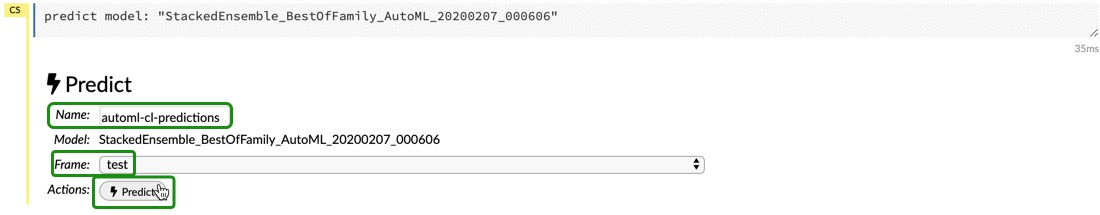Again, now we have several outputs, but now they are the performance on the test set. So we can take a look at the ROC Curve and AUC on the test set, confusion matrix, the maximum metrics at their specific thresholds, the maximum metrics, and the gains/lift table.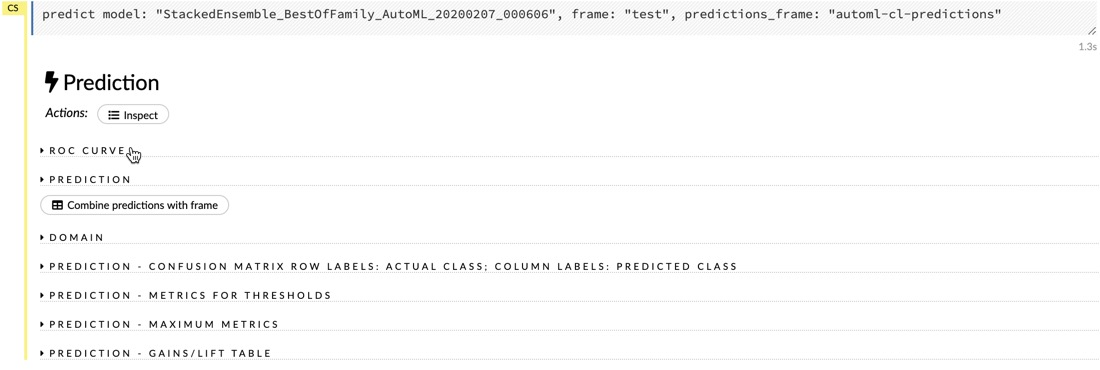Go over the results by yourself, and see how they compare to the results from the cross-validation. Are the results what you expected? Do the scores make sense to you? How are the results from Flow similar to the ones from our Jupyter Notebook? How are they different?

After you are done exploring the results, move on to the next task, where we will do a last run of AutoML for the regression use case.

Scroll up to the Assistance panel once again, and click on runAutoML. We will do something similar to what we just did in the previous task. In the AutoML settings, change Project Name to `flow-automl-regression`, for Training Frame choose `train`, for Response Column choose `ORIGINAL_INTEREST_RATE`, and choose `test` for the Leaderboard Frame.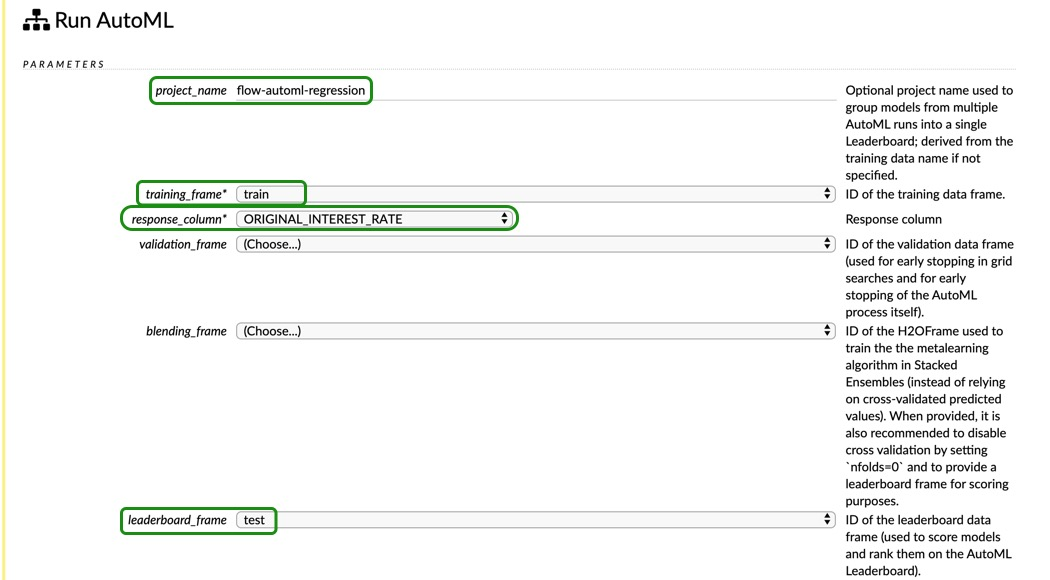for ignore columns select the following:

• `FIRST_PAYMENT_DATE,`
• `MATURITY_DATE,`
• `MORTGAGE_INSURANCE_PERCENTAGE,`
• `PREPAYMENT_PENALTY_MORTGAGE_FLAG,`
• `PRODUCT_TYPE,`
• `LOAN_SEQUENCE_NUMBER,`
• `PREPAID,`
• `DELINQUENT`

Please note that in the figure below, only three columns are selected, but we are ignoring all the above columns, just not showing them in the figure below.

Change the sort metric to `RMSE.` Let's use all the models, so make sure all the models are unchecked, or click None in the exclude_algos menu.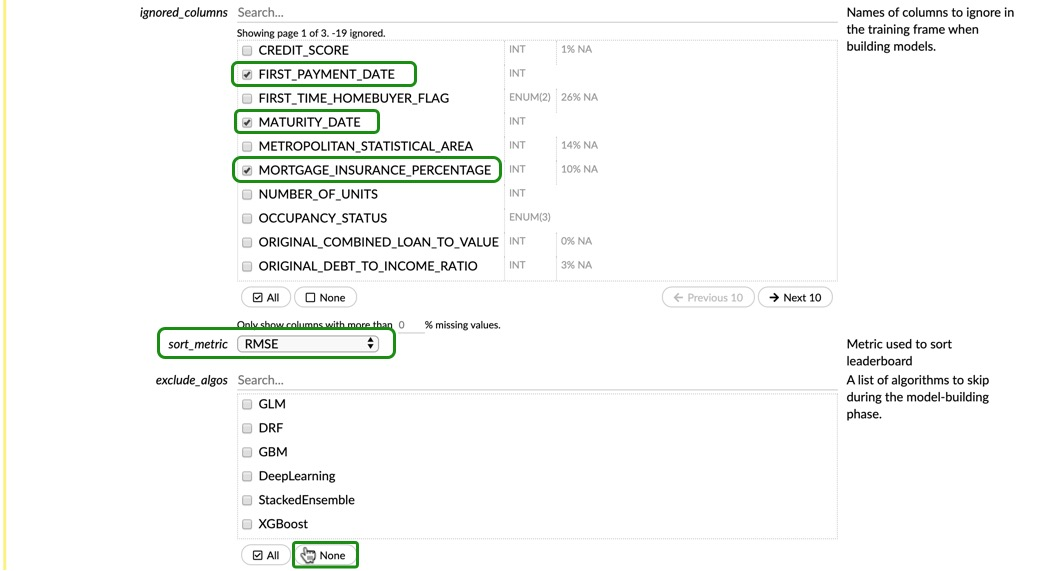Set the Seed to `42,` change the number of max_runtime_secs to `900,` and change the stopping_metric to `RMSE.`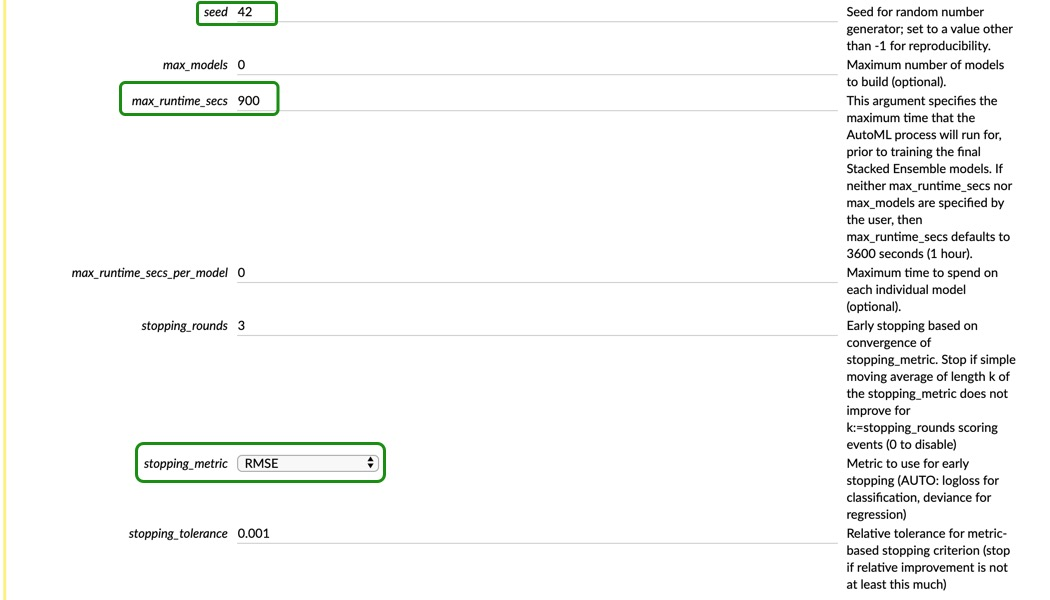Lastly, leave the expert settings as default, and click on Build Model: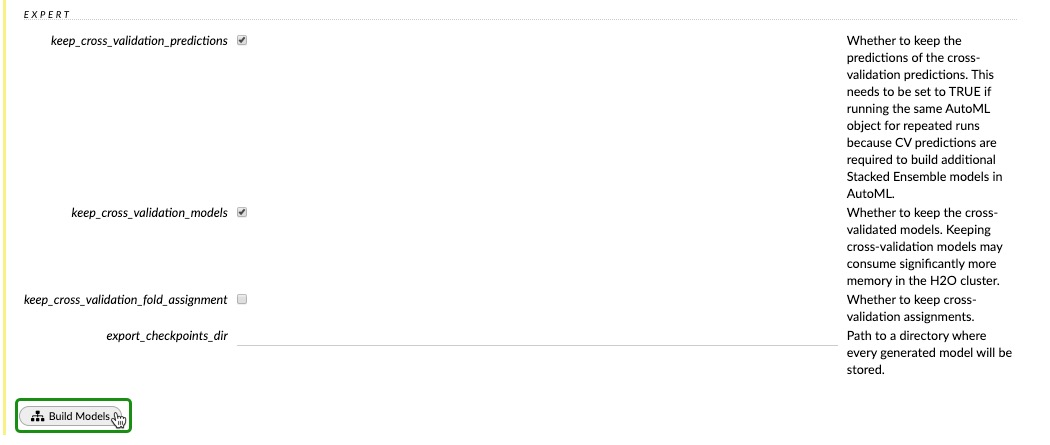Once it's done, click on View and you should be able to see the Leaderboard and the Event Log: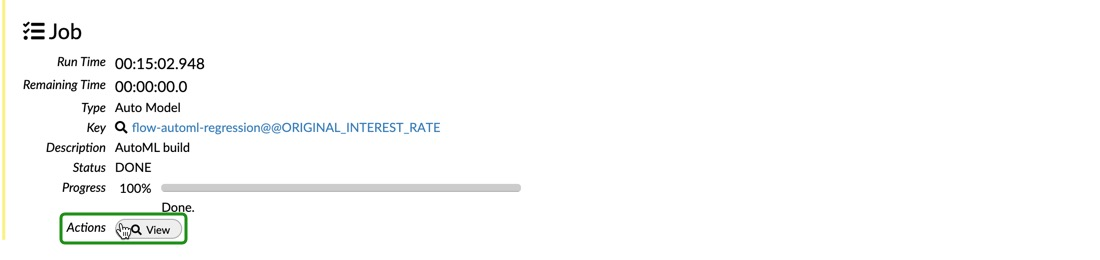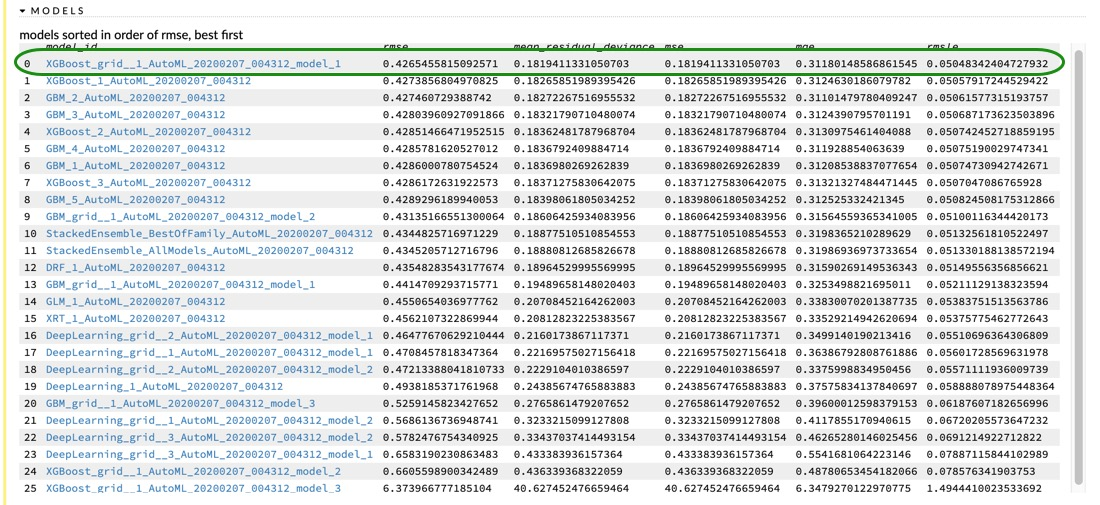In the leaderboard, we can see that the best model from AutoML is an XGBoost, which is different from our AutoML in the Jupyter Notebook; however, the best model from our notebook is still in the top five models.

Click on the best model and explore the results. Take a look at the parameters and their descriptions. If we were trying to explore different models, we could use this model as a baseline and do a local grid search based on the parameters found by the AutoML.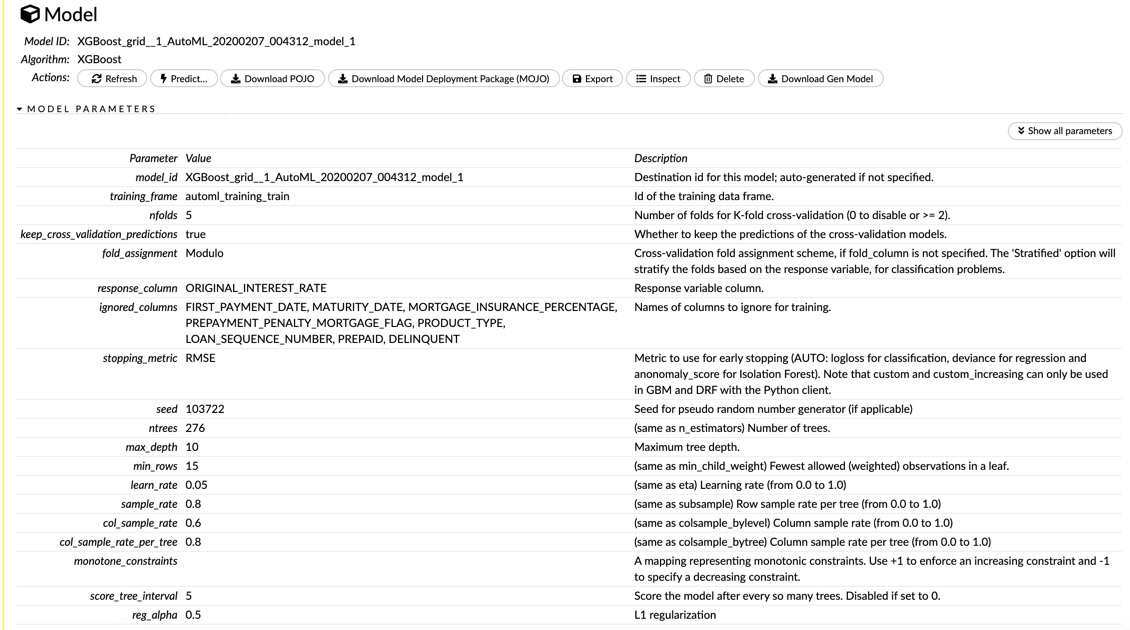You can also look at the scoring history and the variable importance plot (not shown here), and all the outputs related to a regression use case.

Let's make some predictions and explore the results. At the top of the Model panel, click on Predict.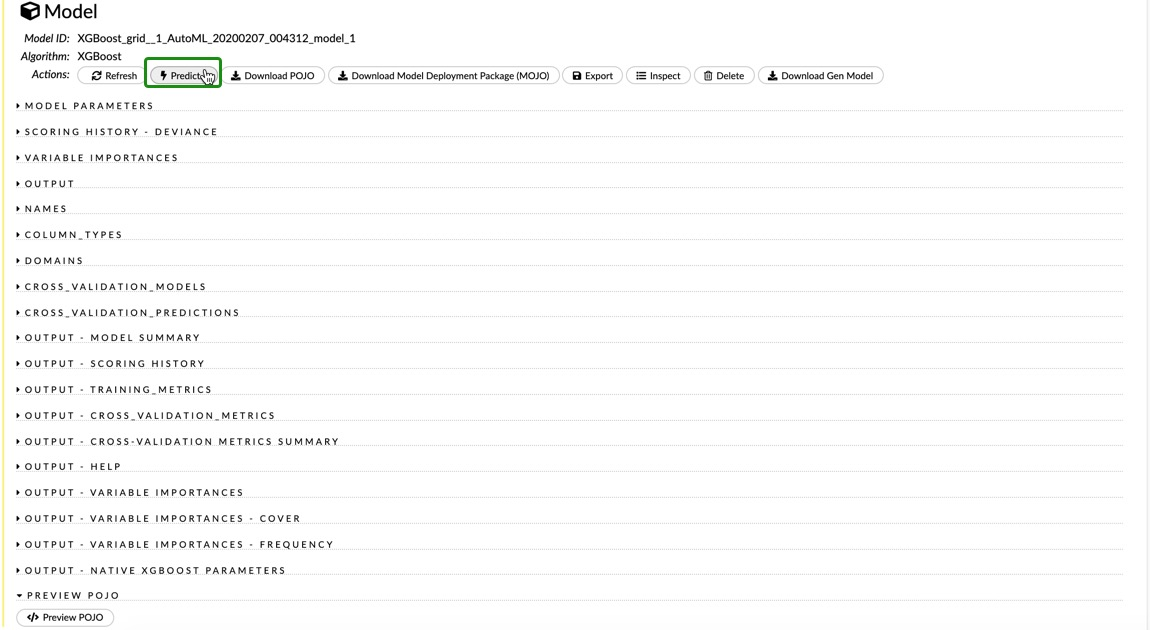Name it `flow-xgb-predictions` and choose `test` for Frame and click on Predict.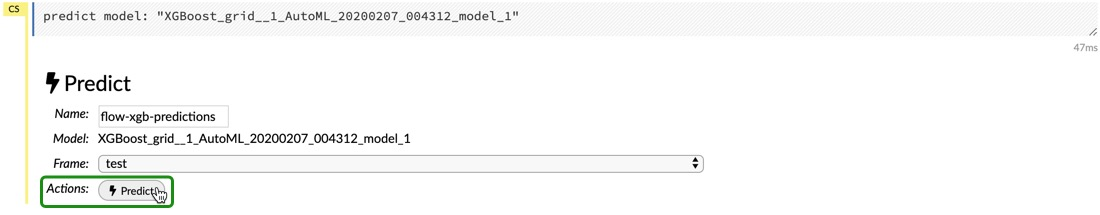Once you're done, you will see a summary of the scores on the test set.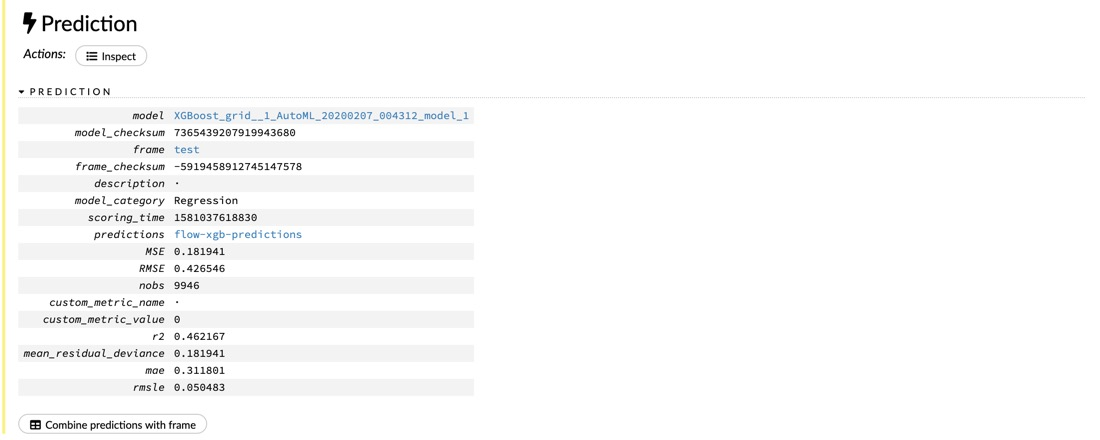If you click on predictions, you will get a summary of another frame. Click on View Data you will get the actual predictions for each sample of the data frame.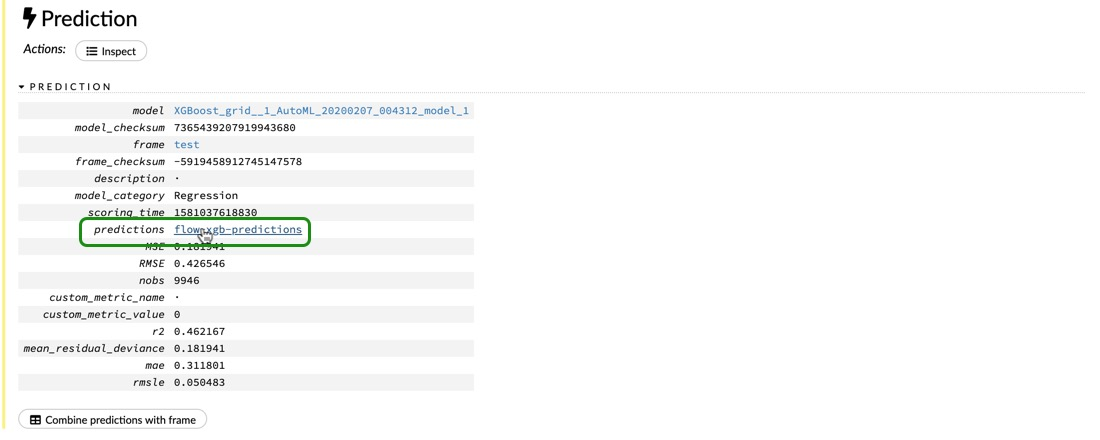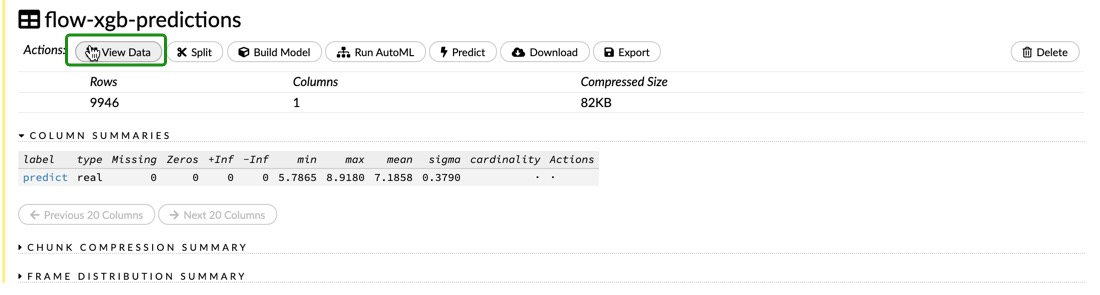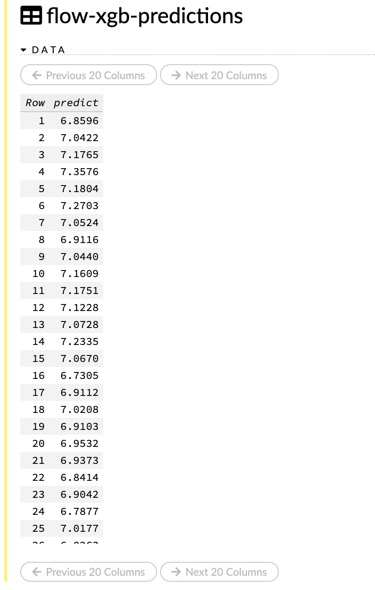Feel free to explore the results on your own. Are the first ten predictions similar to what we got in our Jupyter Notebook? Are the metrics from the test set close to those of the cross-validation?

Now that you can run AutoML in Flow and in the Jupyter Notebook, you can focus on a task and run it for a longer time; you can even run AutoML on the 500k subset of the dataset and see how the results compare to the results in this self-paced course.

If you do not want to attempt the challenge, you can shut down your cluster; otherwise, check out the next task.

``````h2o.cluster().shutdown()
``````

AutoML can help us find the best models faster, and narrow down our search for the parameters for those models. Since you learned to tune some of the most common models in H2O, try to further tune the GBM that we found when we did the regression use-case in our Jupyter Notebook and see how much you can improve the scores. Can you tune the GBM so that it performs better than the XGBoost that we tuned in the previous self-paced course, using a smaller dataset? Give it a try and put your knowledge to practice.

Introduction to machine learning with H2O-3 - Clustering (Unsupervised Learning) coming soon.